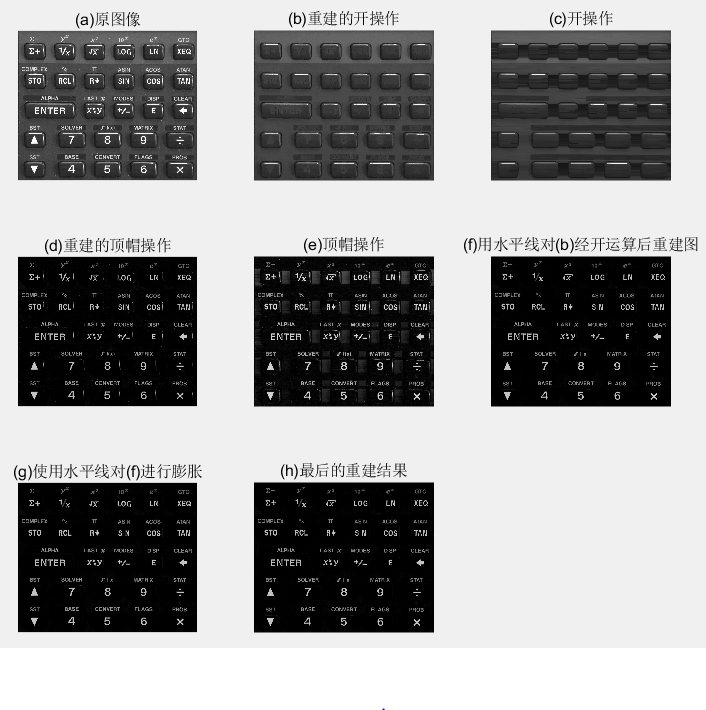# 图像处理找图像对称

## 数字图像处理期中学习报告

2017-12-03 17:28:28 arryCC 阅读数 3945
• ###### 环路后处理-样点自适应滤波(SAO)

掌握视频编解码的基础知识 讲解新一代视频编解码标准HEVC 通俗易懂 知识点非常全面

104人学习 梅奥
免费试看

# 数字图像处理

## 一、学习内容总结

### 1. 第一章 绪论

1. 定义我们称之为数字图像处理领域的范围；
2. 通过考察几个领域，给出图像处理技术状况的概念；
3. 讨论图像处理用到的几种方法；
4. 概述通用目的的典型图像处理系统的组成。

#### 1.1 什么是数字图像处理

• 强度灰度：一幅图像可以被定义为一个二维函数 $f\left(x,y\right)$$f(x,y)$，其中 $x,y$$x,y$ 是空间（平面）坐标，而在任何一处的幅值 $f$$f$ 被称为在该点的灰度或强度。
• 数字图像：当 $x,y$$x,y$ 或灰度值 $f$$f$有限的离散数值时，称该图像为数字图像。也就是说数字图像是由有限数量的元素组成，每个元素都有特定的位置和幅值。这些元素被称为图画元素图像元素像素
• 数字图像处理 : 指用特定的计算机来处理数字图像。

#### 1.2 使用数字图像处理领域的实例

• 伽马射线成像：医学和天文。
• X射线成像：最早用于成像的电磁辐射源之一，医学诊断。
• 紫外波段成像 ：荧光显微镜。
• 可见光及红外线成像 ：可见显微镜技术，遥感，天气预测和预报，红外卫星图像，自动视觉检测，检测丢失的部件，指纹图像。
• 微波波段成像 ：雷达。
• 无线电波段成像 ：天文学和医学（核磁共振）。
• 其他方式 ：声波成像，电子显微镜方法，（由计算机产生的）合成图像。

#### 1.3 数字图像处理的基本步骤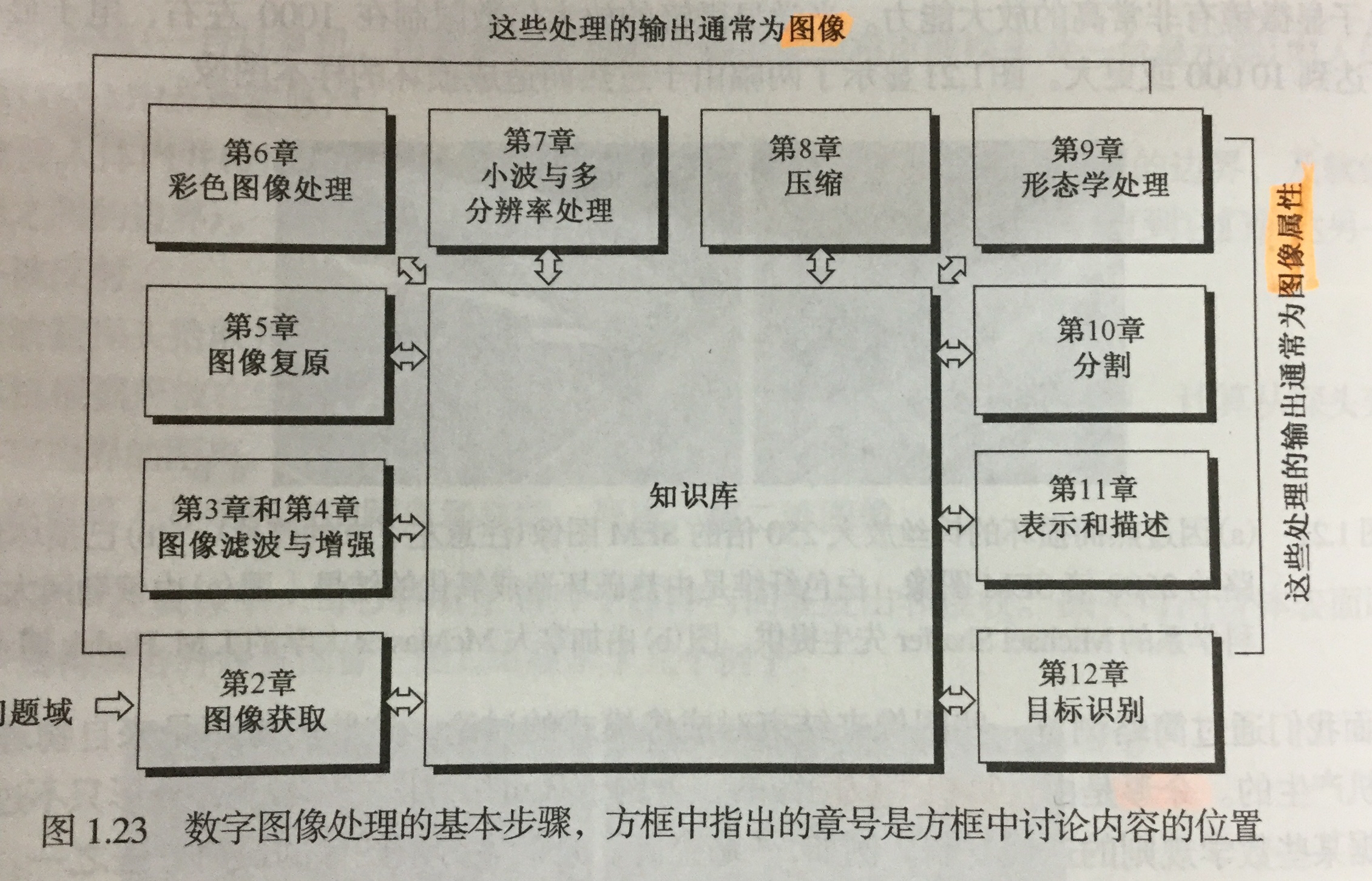#### 1.4 图像处理系统的组成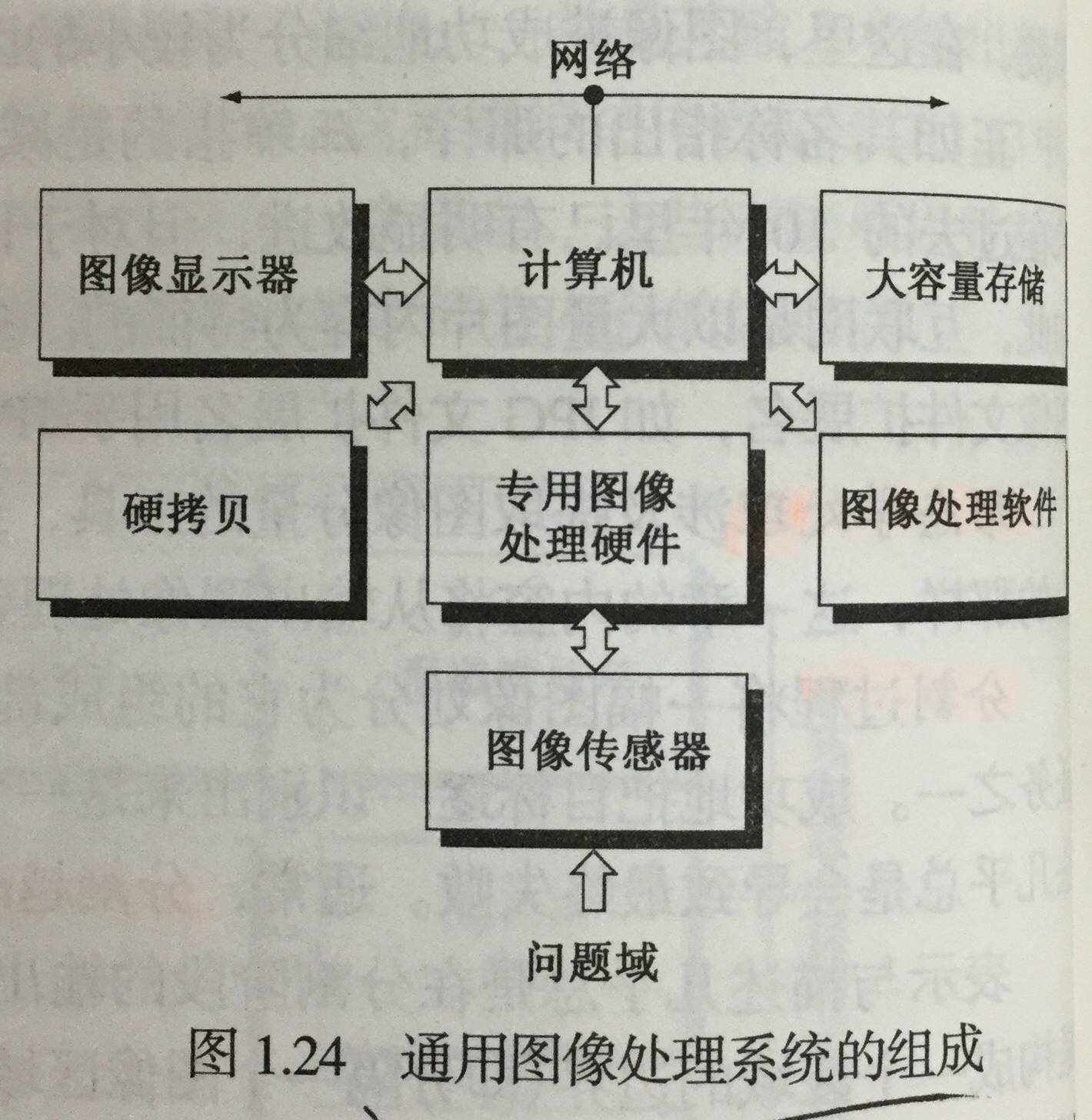### 2. 第二章 数字图像处理基础

#### 2.1 视觉感知要素

• 人眼的结构

重点介绍视网膜里的两类光感受器

• 锥状体 ：对颜色高度敏感，这种视觉称为白昼视觉或者亮视觉。高照明水平下执行。
• 杆状体 ：没有彩色感觉，对低照明度敏感，称为暗视觉或微光视觉。低照明水平下执行。
• 亮度适应与辨别

• 亮度适应现象 ：视觉系统不能同时在一个范围内工作，它是通过改变其整个灵敏度来完成这一较大变动的。
• 韦伯比 ：较大：亮度辨别能力较差；反之，较好。
• 感知亮度 不是简单的强度的函数

• 视觉系统往往会在不同强度区域的边界处出现“下冲”或“上冲”现象。
• 同时对比、错觉

#### 2.2 光和电磁波谱

• 电磁波是能量的一种，任何有能量的物体都会释放电磁波谱。它可以用 波长 ($\lambda$$\lambda$)、频率($v$$v$)或能量($E$$E$) 来描述，其中

$\lambda =c/v$

$E=hv$

• 光是一种特殊的电磁辐射，可以被人眼感知。
• 单色光 是没有颜色的光，也成为无色光。唯一属性就是它的强度或者大小，用 灰度级 来表示。单色图像常被称为 灰度图像
• 彩色光源 的质量可以用发光强度、光通量和亮度 来表示。

#### 2.3 图像感知和获取

• 图像获取方式

• 使用单个传感器来获取图像
• 使用条带传感器获取图像
• 使用传感器阵列获取图像
• 简单的图像形成模型

用形如 $f\left(x,y\right)$$f(x,y)$ 的二维函数来表示图像，那么：

$0

$f\left(x,y\right)$$f(x,y)$ 可以用两个分量来表征：

• 入射分量 入射到被观察场景的光源照射总量，用$i\left(x,y\right)$$i(x,y)$ 表示;
• 反射分量 场景中物体所反射的光照总量，用$r\left(x,y\right)$$r(x,y)$ 表示。

所以有：

$f\left(x,y\right)=i\left(x,y\right)r\left(x,y\right),0

#### 2.4 图像取样和量化

• 取样和量化的基本概念

• 取样 ：对坐标值进行数字化
• 量化 ： 对幅值数字化

数字图像的质量在很大程度上取决于取样和量化中所用的样本数灰度级

• 数字图像表示

用数列矩阵来表示一幅数字图像。在实数矩阵中，每个元素称为图像单元、图像元素或像素。

对比度 一幅图像最高和最低灰度级间的灰度差为对比度。

存储数字图像所用的比特数为：

$b=M×N×k,当M=N时，b={N}^{2}k$

灰度级数$L={2}^{k}$$L = 2^k$

• 空间和灰度分辨率

• 空间分辨率 ：图像中可辨别的最小细节的度量。在数量上，表示每单位距离线对数和每单位距离点数是最通用的度量（必须针对空间单位来规定才有意义）。
• 灰度分辨率 ：指在灰度级中可分辨的最小变化。
• 图像内插

用已知数据来估计未知位置的数据处理。是基本的图像重取样方法。可以处理图像的放大和缩小。

#### 2.5 像素间的基本关系

• 相邻像素

位于坐标 $\left(x,y\right)$$(x,y)$ 处的像素 $p$$p$ 有4个水平和垂直上的相邻像素，用 ${N}_{4}\left(p\right)$$N_4(p)$ 表示；有四个对角相邻像素，用 ${N}_{D}\left(p\right)$$N_D(p)$ 表示。如果 $p$$p$ 位于图像边界，则某些邻点可能 落在图像外边。

• 邻接性、连通性、区域和边界

• 4邻接、8邻接、混合邻接
• 距离度量

• 欧氏距离(圆)
• ${D}_{4}$$D_4$ 城市街区距离(菱形)
• 棋盘距离(正方形)

#### 2.6 常用数学工具介绍

• 阵列和矩阵操作
• 线性操作和非线性操作
• 算术操作
• 集合和逻辑操作
• 基本集合操作
• 逻辑操作
• 模糊集合
• 空间操作
• 单像素操作
• 邻域操作
• 几何空间变换与图像配准
• 向量和矩阵操作
• 图像变换

### 3.第三章

#### 3.1 背景知识

• 空间域 就是简单的包含图像像素的平面。空间域处理可用以下方式表示：

$g\left(x,y\right)=T\left[f\left(x,y\right)\right],T是在点\left(x,y\right)的邻域上处定义的一种算子$

• 灰度变换函数
$s=T\left(r\right),r,s分别代表处理前后的像素值$

#### 3.2 基本灰度变换函数

• 图像反转

得到灰度范围为 $\left[0,L-1\right]$$[0,L-1]$ 的一幅图像的反转图像:（得到等效的照片底片）

$s=L-1-r$

• 对数变换

对数变换的通用形式：

$s=clog\left(1+r\right)$

扩展图像中暗像素的值，同时压缩更高灰度级的值。反对数变换的作用与此相反。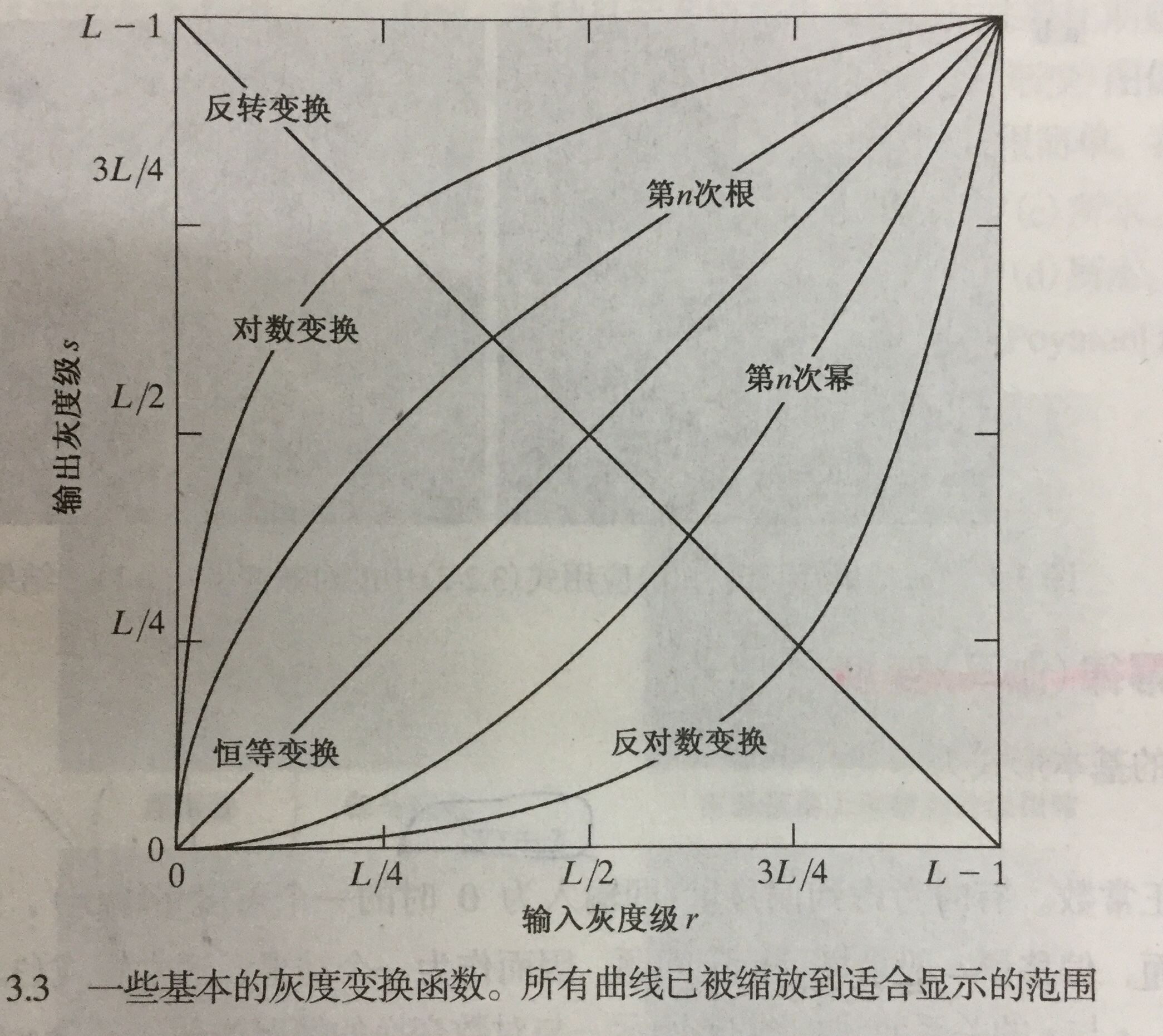• 幂律变换(伽马)变换

基本形式：

$s=c{r}^{\gamma }$

$\gamma <1$$\gamma < 1$ 变亮，大于1变暗，$c=\gamma =1$$c = \gamma = 1$ 恒等变换。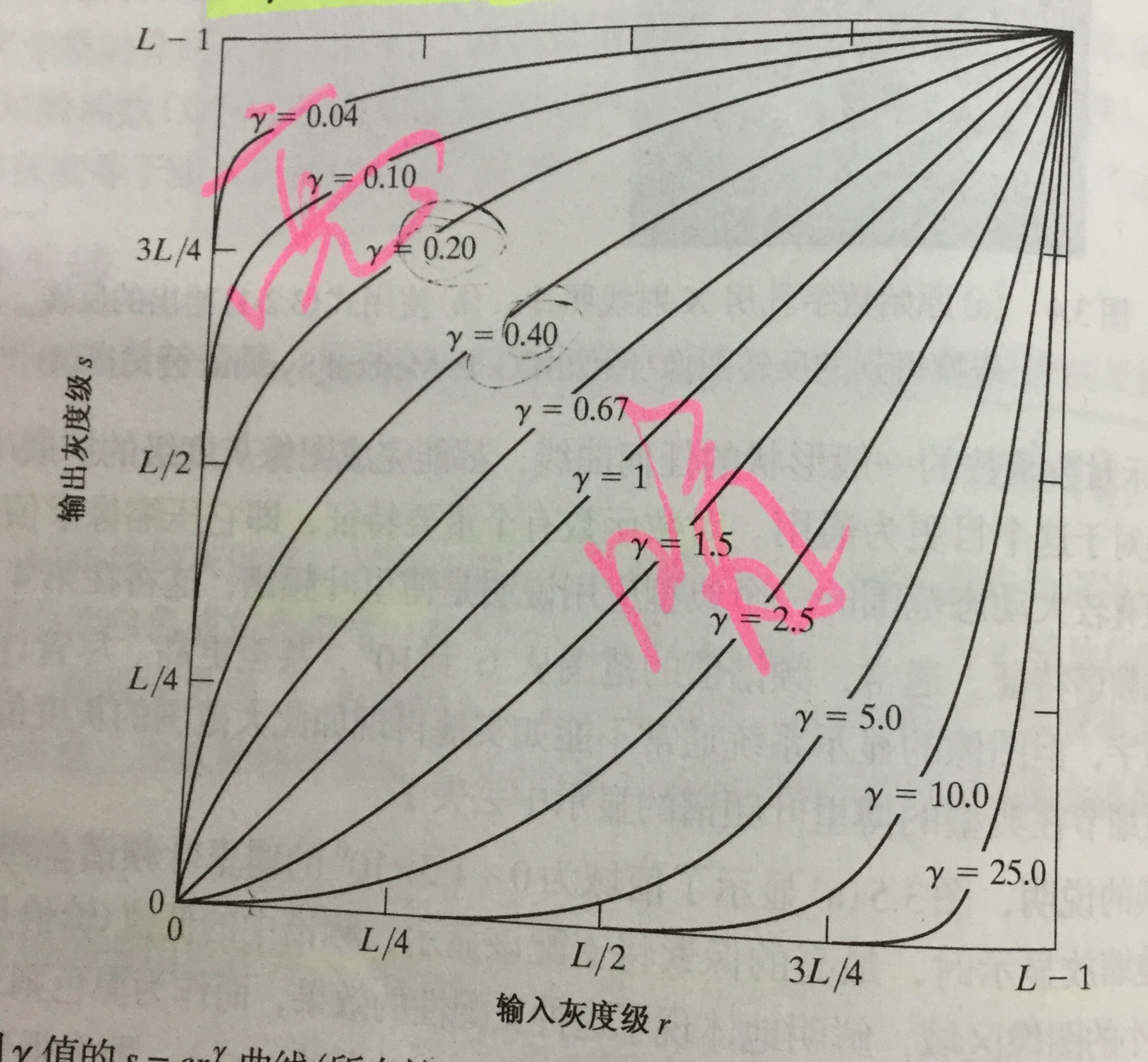* 分段线性变换函数
* 对比度拉伸：扩展图像灰度级动态范围处理，因此它可以跨越记录介质和显示装置的全部灰度范围。

根据$r,s$ 的取值，变换可以为线性函数和阈值处理函数。

• 灰度级分层：突出特定图像灰度范围的亮度。有两种方法：

• 突出范围 $\left[A,B\right]$$[A,B]$ 内的灰度，并将所有其他灰度降低到一个更低的级别；
• 突出范围$\left[A,N\right]$$[A,N]$ 内的灰度，并保持所有其他灰度级不变。
• 比特平面分层：突出特定比特为整个图像外观作贡献。

• 4个高阶比特平面，特别是最后两个比特平面，包含了在视觉上很重要的大多数数据。
• 低阶比特平面在图像中贡献更精细的灰度细节。

得出结论：储存四个高阶比特平面将允许我们以可接受的细节来重建原图像。这样可减少50%的存储量。

#### 3.3 直方图的处理

• 理论基础：若一幅图像的像素倾向于占据可能的灰度级并且分布均匀，则该图像会有高对比度的外观并展示灰色调的较大变化。

• 直方图均衡：

• 灰度范围为 $\left[0,L-1\right]$$[0,L-1]$ 的数字图像的直方图是离散函数 $h\left({r}_{k}\right)={n}_{k}$$h(r_k) = n_k$,其中 ${r}_{k}$$r_k$ 是第 $k$$k$ 级灰度值，${n}_{k}$$n_k$ 是图像中灰度为${r}_{k}$$r_k$ 的像素的个数。
• 通过转换函数$T\left({r}_{k}\right)$$T(r_k)$变换，得到直方图均衡化。
• 应用：自适应对比度增强。
• 直方图匹配：用于处理后有特殊直方图的方法。

• 局部直方图处理：以图像中每个像素邻域中的灰度分布为基础设计变换函数，来增强图像中小区域的细节。

• 在图像增强中使用直方图统计：提供这样一种增强图像的方法：

在仅处理均值和方差时，实际上直接从取样值来估计它们，不必计算直方图。这些估计被称为取样均值和取样方差。

#### 3.4 空间滤波基础

• 空间滤波机理

• 空间滤波器的组成：
• 一个邻域
• 对该邻域包围的图像像素执行的预定义操作

滤波产生的是一个新像素，新像素的坐标等于邻域中心的坐标，像素的值是滤波操作的结果。

• 空间相关与卷积

• 相关：滤波器模板移过图像并计算每个位置乘积之和的处理。一个大小为$m×n$$m×n$ 的滤波器与一幅图像 $f\left(x,y\right)$$f(x,y)$ 做相关操作，可表示为$w\left(x,y\right)☆f\left(x,y\right)$$w(x,y)☆f(x,y)$
• 卷积：与相关机理相似，但滤波器首先要旋转${180}^{o}$$180^o$ 一个大小为$m×n$$m×n$ 的滤波器与一幅图像 $f\left(x,y\right)$$f(x,y)$ 做j卷积操作，可表示为$w\left(x,y\right)★f\left(x,y\right)$$w(x,y)★f(x,y)$

#### 3.5 平滑空间滤波器

• 平滑线性滤波器(均值滤波器)

它使用滤波器确定的邻域内像素的平均灰度值代替图像中每个像素的值。应用：

• 降低噪声
• 灰度级数量不足而引起的伪轮廓效应的平滑处理
• 去除图像的不相关细节
• 统计排序(非线性)滤波器

最有代表性的是中值滤波器 ，特点：

• 将像素邻域内灰度的中值(在中值计算中，包括原像素值)代替该像素的值；
• 对处理脉冲噪声(椒盐噪声)非常有效。

#### 3.6 锐化空间滤波器

• 拉普拉斯算子：最简单的各向同性微分算子，是一个线性算子。因其为微分算子，因此强调的是图像中灰度的 突变而不是灰度级缓慢变换的区域。

• 非锐化隐蔽和高提升滤波：从原图像中减去一部分非锐化的版本。步骤：

• 模糊原图像
• 从原图像减去模糊图像
• 将模板加到原图像上
• 梯度：图像处理中的一阶微分用梯度实现。对于函数$f\left(x\right)$$f(x)$ ，在坐标$\left(x,y\right)$$(x,y)$ 处的梯度定义为二维列向量。它指出在位置$f\left(x,y\right)$$f(x,y)$$f$$f$的最大变化率方向。

应用：边缘增强。

### 4.第四章

#### 4.1. 基本概念

• 傅里叶概念：任何周期函数都可以表示为不同频率的正弦和或余弦和的形式，每个正弦项和或余弦项乘以不同的系数（傅里叶级数）。
• 傅里叶变换：在非周期函数用正弦和或余弦和乘以加权函数的积分来表示的公式。
• 介绍复数、傅里叶级数、冲击及其取样特征、连续函数的傅里叶变换以及之前提过的卷积。

#### 4.2. 取样与取样函数中的傅里叶变换

• 取样

在连续函数$f\left(x,y\right)$$f(x,y)$ 中模拟取样的一种方法是：用一个$\mathrm{\Delta }T$$\Delta T$ 单位间隔的冲击串作为取样函数去乘以$f\left(t\right)$$f(t)$ .

• 取样函数的傅里叶变换

空间域来两个函数乘积的傅里叶变换是两个函数在频率域的卷积。

• 取样定理

如果以超过函数最高频率的两倍的取样来获取样本，连续的带限函数可以完全从它的样本集来恢复。

#### 4.3. DFT小结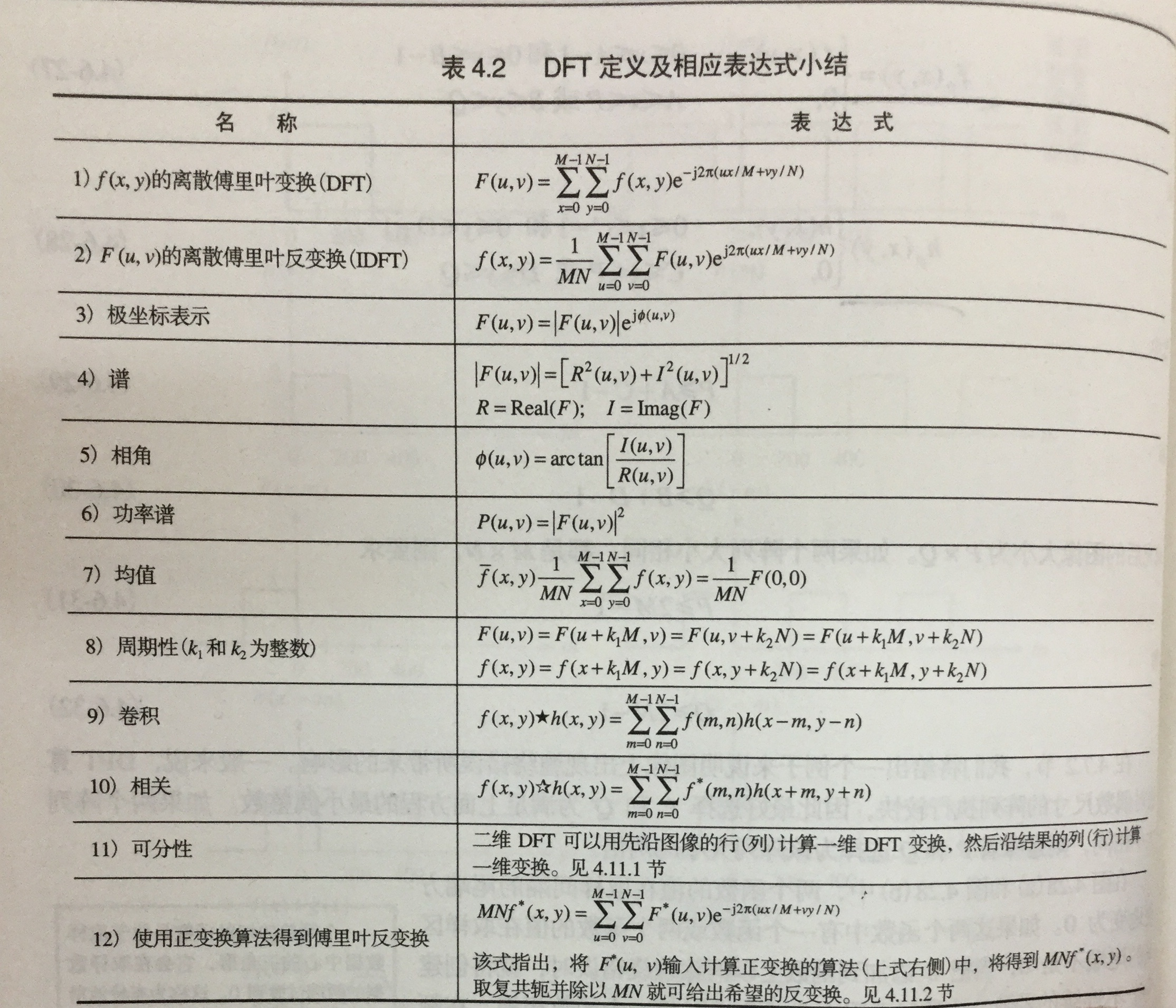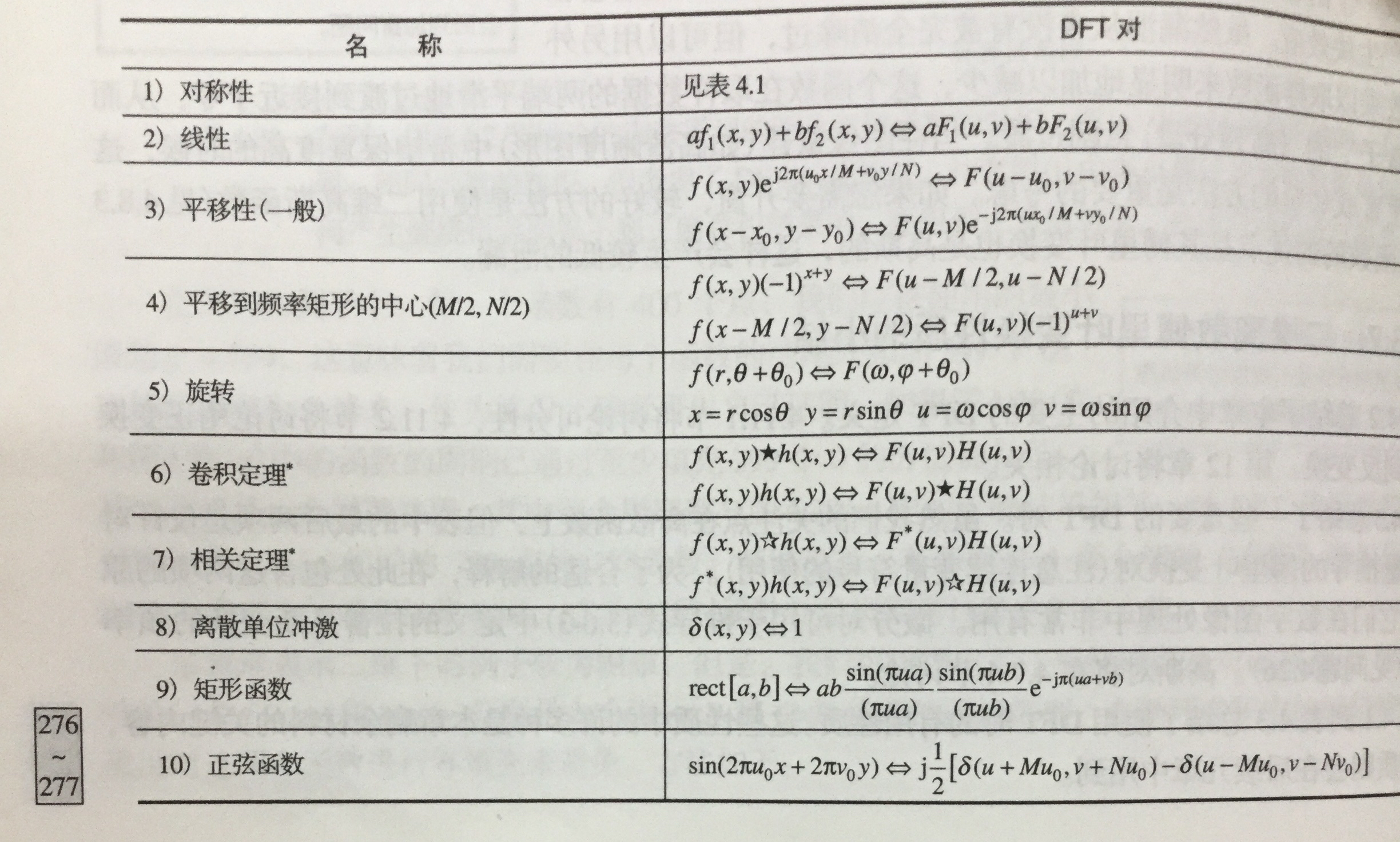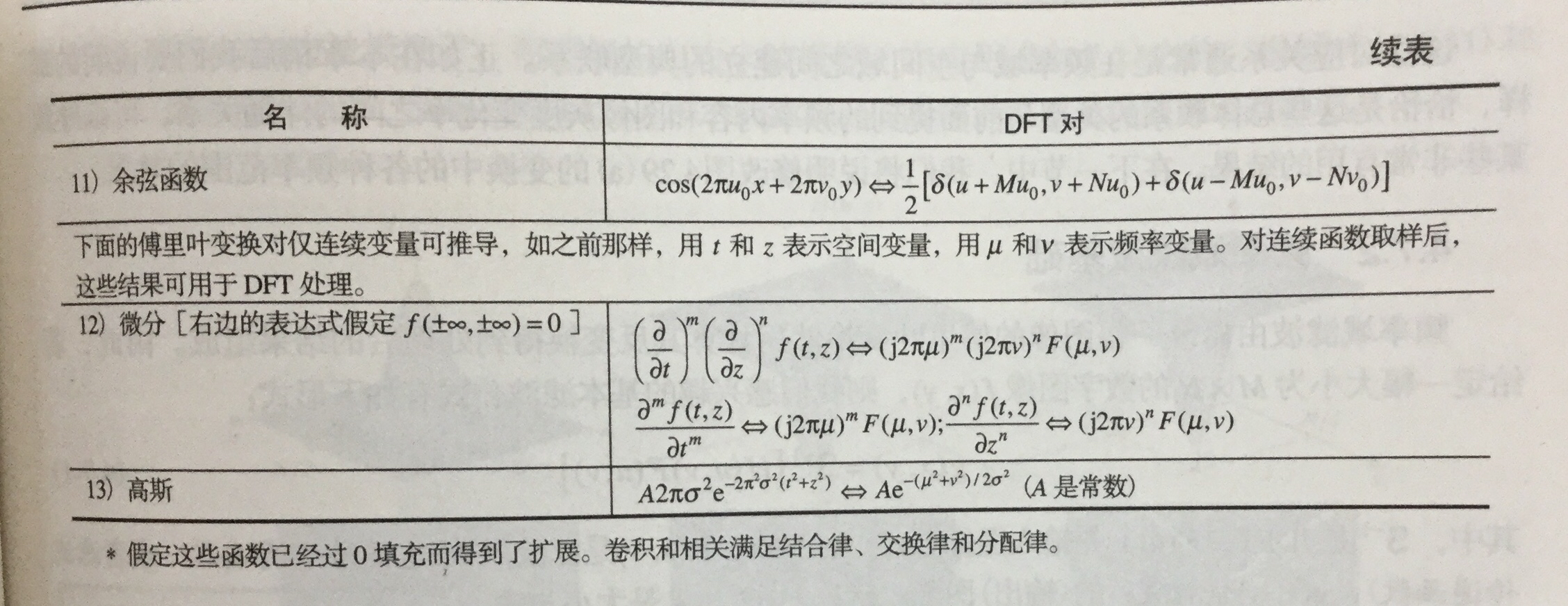#### 4.4. 频率域滤波

• 步骤
• 等到填充参数$P$$P$$Q$$Q$
• 形成大小为$P×Q$$P × Q$ 的填充后的图像${f}_{p}\left(x,y\right)$$f_p(x,y)$
• $\left(-1{\right)}^{x+y}$$(-1)^{x+y}$ 乘以${f}_{p}\left(x,y\right)$$f_p(x,y)$移到其变换中心
• 计算上一步骤的DTF，得到$F\left(u,v\right)$$F(u,v)$
• 生成实的、对称的滤波函数$H\left(u,v\right)$$H(u,v)$
• 得到处理后的图像${g}_{p}\left(x,y\right)$$g_p(x,y)$
• ${g}_{p}\left(x,y\right)$$g_p(x,y)$ 的做上限提取$M×N$$M×N$区域 ，得到最终的处理结果$g\left(x,y\right)$$g(x,y)$
• 空间域与频率域间的纽带是卷积定理。

#### 4.5. 使用频率域滤波器平滑图像

• 定义总结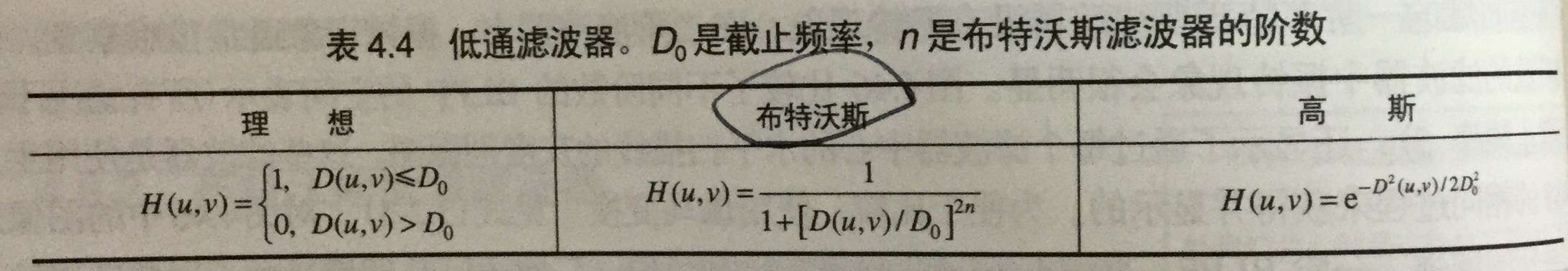• 特性
• 理想低通滤波器(ILFP)
• 特性：模糊和振铃。
• 布特沃斯低通滤波器(BLPF)
• 特性：随着阶数增高，其振铃和负值变明显。(一阶时无)
• 高斯低通滤波器(GLPF)
• 特性：无振铃

#### 4.6. 使用频率域滤波器锐化图像

• 三种高通滤波器来锐化图像
• 定义总结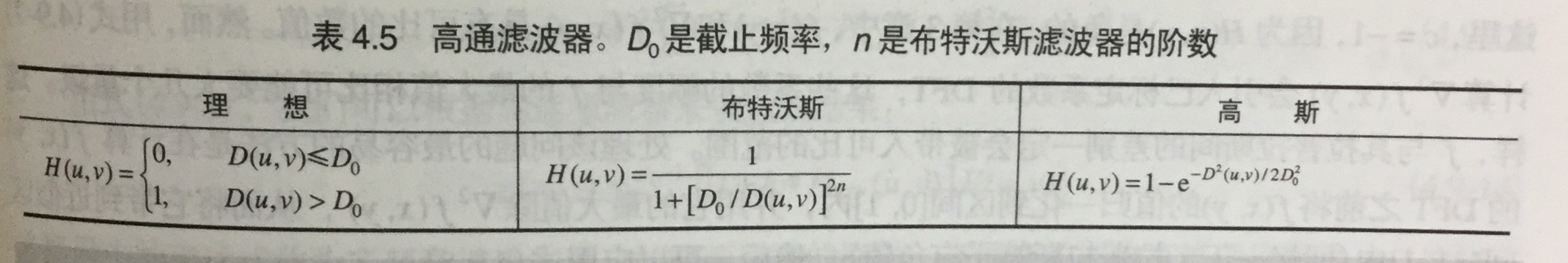* 特性

* 理想高通滤波器(IHPF)
* 有振铃
* 布特沃斯高通滤波器(BHPF)
* 比IHPF更平滑
* 高斯低通滤波器(GHPF)
* 比前两个更平滑，即使微小物体和细线条得到的结果也比较其清晰

• 其他方式

• 拉普拉斯算子
• 钝化模板、高提升滤波和高频强调滤波
• 同态滤波

#### 4.7.选择性滤波器

• 带阻滤波器和带通滤波器

• 带阻滤波器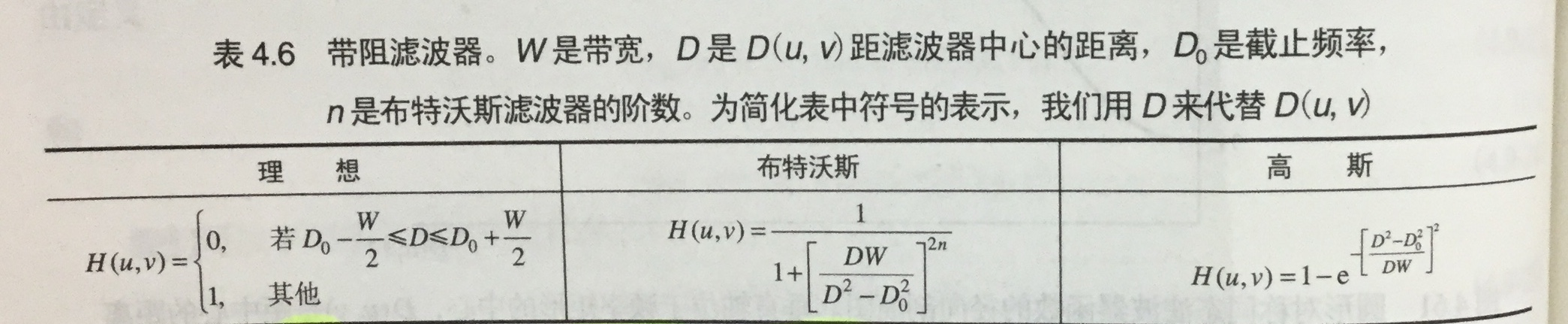• 带通滤波器

• 通过1减去带阻得到。

• 陷波滤波器：拒绝事先定义的关于频率矩形中心的一个邻域的频率。

• 陷波带阻滤波器

用中心已被平移到陷波滤波中心的高通滤波器的乘积来构造。

• 陷波带通滤波器

通过1减去带阻得到。

## 图像处理中的傅里叶变换

2017-04-15 19:34:01 m0_37264397 阅读数 61094
• ###### 环路后处理-样点自适应滤波(SAO)

掌握视频编解码的基础知识 讲解新一代视频编解码标准HEVC 通俗易懂 知识点非常全面

104人学习 梅奥
免费试看

## [Python图像处理] 二十二.Python图像傅里叶变换原理及实现

2019-04-23 16:24:29 Eastmount 阅读数 19610
• ###### 环路后处理-样点自适应滤波(SAO)

掌握视频编解码的基础知识 讲解新一代视频编解码标准HEVC 通俗易懂 知识点非常全面

104人学习 梅奥
免费试看

PS：请求帮忙点个Star，哈哈，第一次使用Github，以后会分享更多代码，一起加油。

1.图像傅里叶变换
2.Numpy实现傅里叶变换
3.Numpy实现傅里叶逆变换
4.OpenCV实现傅里叶变换
5.OpenCV实现傅里叶逆变换

PS：文章参考自己以前系列图像处理文章及OpenCV库函数，同时参考如下文献：
《数字图像处理》（第3版），冈萨雷斯著，阮秋琦译，电子工业出版社，2013年.
《数字图像处理学》（第3版），阮秋琦，电子工业出版社，2008年，北京.
《OpenCV3编程入门》，毛星云，冷雪飞，电子工业出版社，2015，北京.

tenderwx-数字图像处理-傅里叶变换在图像处理中的应用

# 一.图像傅里叶变换原理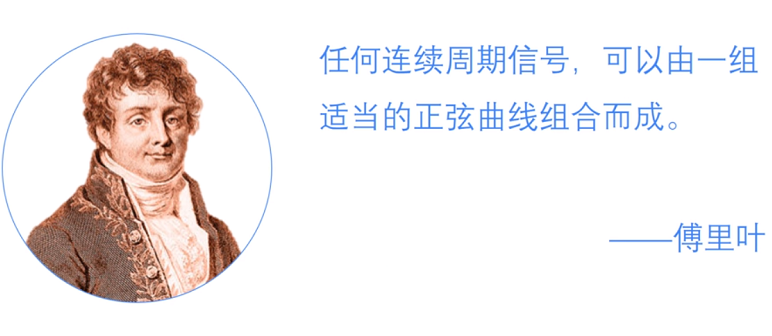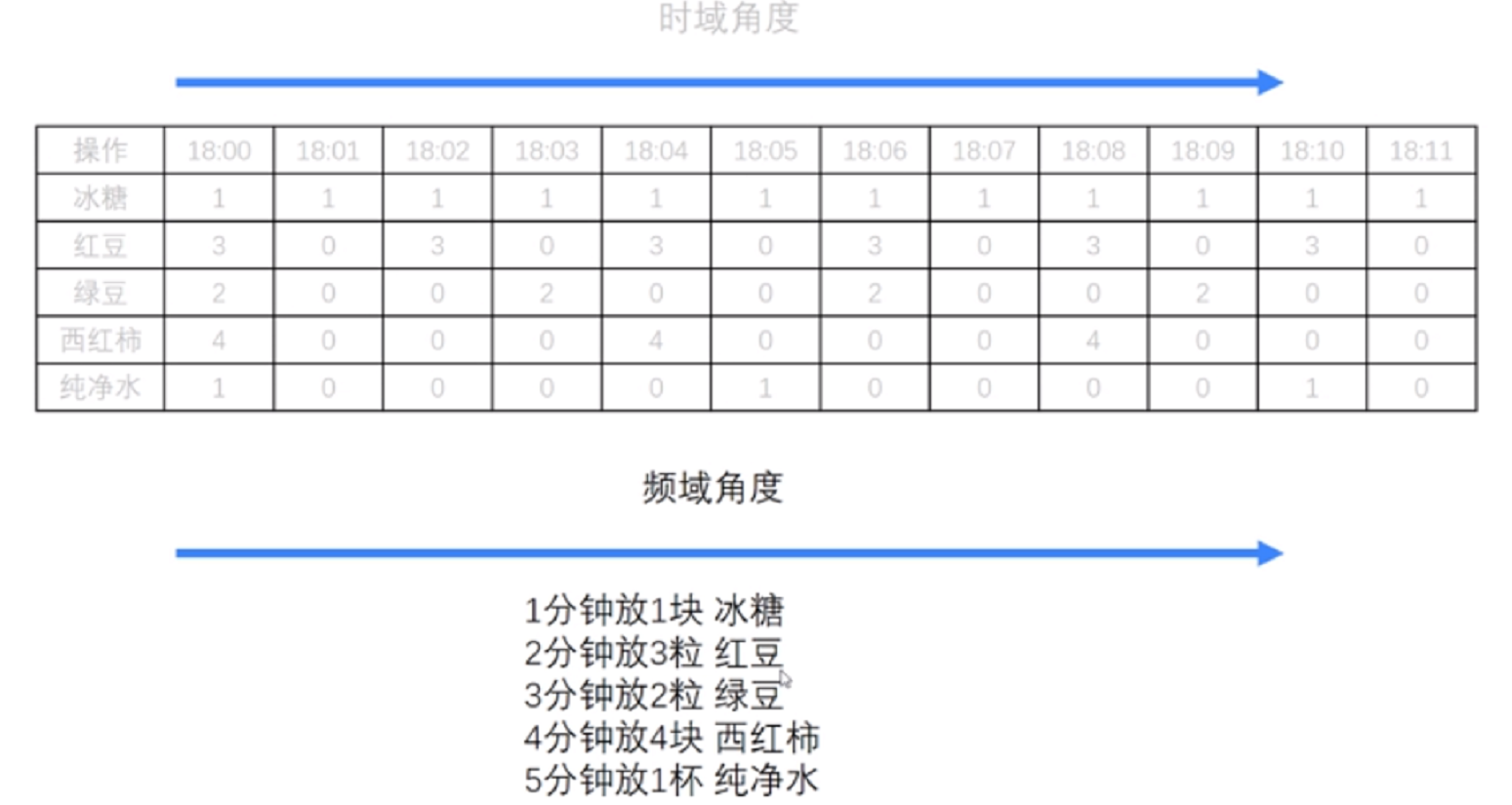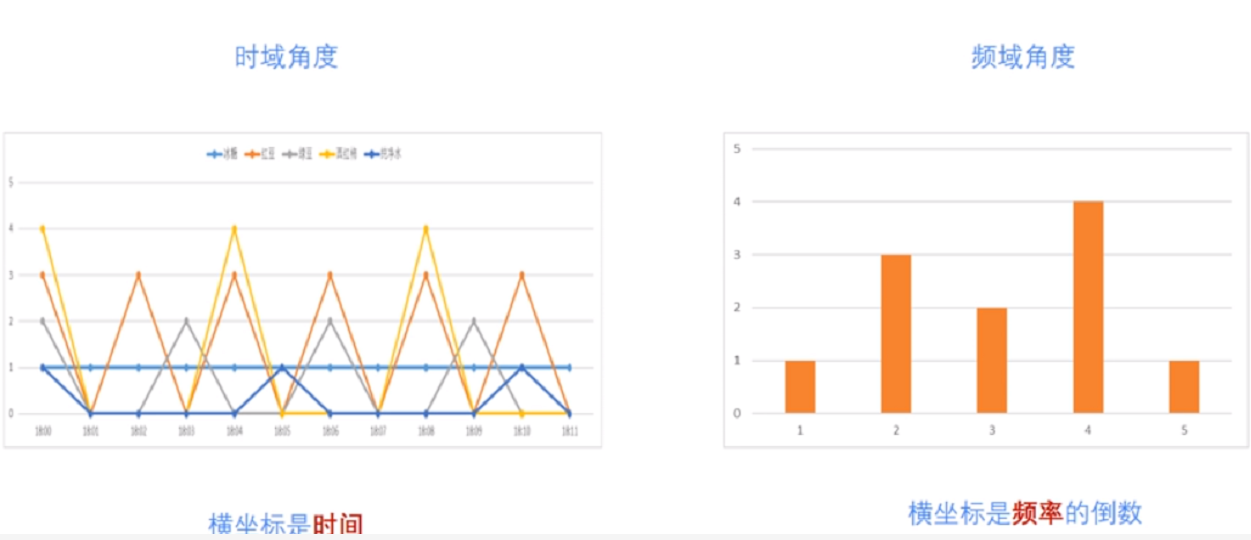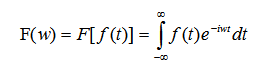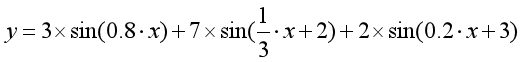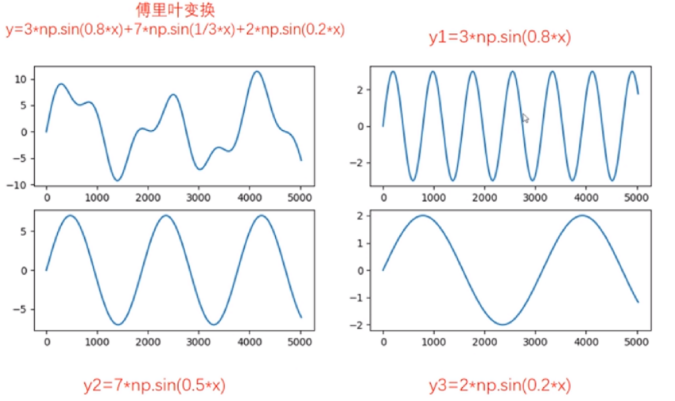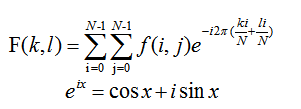# 二.Numpy实现傅里叶变换

Numpy中的 FFT包提供了函数 np.fft.fft2()可以对信号进行快速傅里叶变换，其函数原型如下所示，该输出结果是一个复数数组（Complex Ndarry）。

fft2(a, s=None, axes=(-2, -1), norm=None)

• a表示输入图像，阵列状的复杂数组
• s表示整数序列，可以决定输出数组的大小。输出可选形状（每个转换轴的长度），其中s表示轴0，s表示轴1。对应fit(x,n)函数中的n，沿着每个轴，如果给定的形状小于输入形状，则将剪切输入。如果大于则输入将用零填充。如果未给定’s’，则使用沿’axles’指定的轴的输入形状
• axes表示整数序列，用于计算FFT的可选轴。如果未给出，则使用最后两个轴。“axes”中的重复索引表示对该轴执行多次转换，一个元素序列意味着执行一维FFT
• norm包括None和ortho两个选项，规范化模式（请参见numpy.fft）。默认值为无

Numpy中的fft模块有很多函数，相关函数如下：

#计算一维傅里叶变换
numpy.fft.fft(a, n=None, axis=-1, norm=None)
#计算二维的傅里叶变换
numpy.fft.fft2(a, n=None, axis=-1, norm=None)
#计算n维的傅里叶变换
numpy.fft.fftn()
#计算n维实数的傅里叶变换
numpy.fft.rfftn()
#返回傅里叶变换的采样频率
numpy.fft.fftfreq()
#将FFT输出中的直流分量移动到频谱中央
numpy.fft.shift()

# -*- coding: utf-8 -*-
import cv2 as cv
import numpy as np
from matplotlib import pyplot as plt

#读取图像

#快速傅里叶变换算法得到频率分布
f = np.fft.fft2(img)

#默认结果中心点位置是在左上角,
#调用fftshift()函数转移到中间位置
fshift = np.fft.fftshift(f)

#fft结果是复数, 其绝对值结果是振幅
fimg = np.log(np.abs(fshift))

#展示结果
plt.subplot(121), plt.imshow(img, 'gray'), plt.title('Original Fourier')
plt.axis('off')
plt.subplot(122), plt.imshow(fimg, 'gray'), plt.title('Fourier Fourier')
plt.axis('off')
plt.show()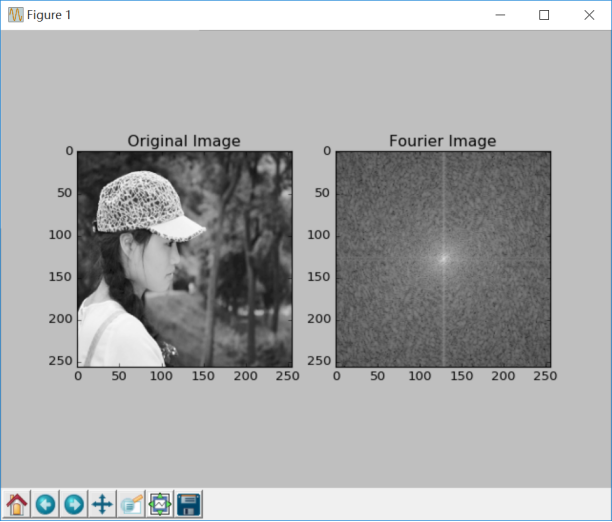# 三.Numpy实现傅里叶逆变换

#实现图像逆傅里叶变换，返回一个复数数组
numpy.fft.ifft2(a, n=None, axis=-1, norm=None)
#fftshit()函数的逆函数，它将频谱图像的中心低频部分移动至左上角
numpy.fft.fftshift()
#将复数转换为0至255范围
iimg = numpy.abs(逆傅里叶变换结果)

# -*- coding: utf-8 -*-
import cv2 as cv
import numpy as np
from matplotlib import pyplot as plt

#读取图像

#傅里叶变换
f = np.fft.fft2(img)
fshift = np.fft.fftshift(f)
res = np.log(np.abs(fshift))

#傅里叶逆变换
ishift = np.fft.ifftshift(fshift)
iimg = np.fft.ifft2(ishift)
iimg = np.abs(iimg)

#展示结果
plt.subplot(131), plt.imshow(img, 'gray'), plt.title('Original Image')
plt.axis('off')
plt.subplot(132), plt.imshow(res, 'gray'), plt.title('Fourier Image')
plt.axis('off')
plt.subplot(133), plt.imshow(iimg, 'gray'), plt.title('Inverse Fourier Image')
plt.axis('off')
plt.show()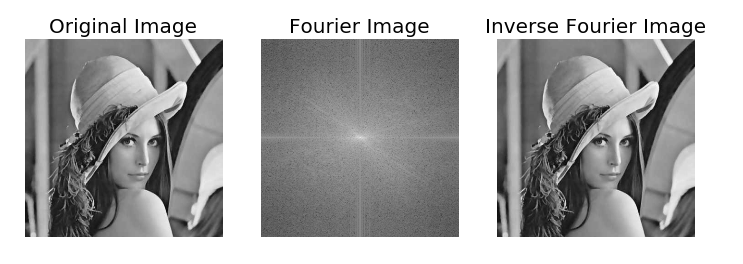# 四.OpenCV实现傅里叶变换

OpenCV 中相应的函数是cv2.dft()和用Numpy输出的结果一样，但是是双通道的。第一个通道是结果的实数部分，第二个通道是结果的虚数部分，并且输入图像要首先转换成 np.float32 格式。其函数原型如下所示：

dst = cv2.dft(src, dst=None, flags=None, nonzeroRows=None)

• src表示输入图像，需要通过np.float32转换格式
• dst表示输出图像，包括输出大小和尺寸
• flags表示转换标记，其中DFT _INVERSE执行反向一维或二维转换，而不是默认的正向转换；DFT _SCALE表示缩放结果，由阵列元素的数量除以它；DFT _ROWS执行正向或反向变换输入矩阵的每个单独的行，该标志可以同时转换多个矢量，并可用于减少开销以执行3D和更高维度的转换等；DFT _COMPLEX_OUTPUT执行1D或2D实数组的正向转换，这是最快的选择，默认功能；DFT _REAL_OUTPUT执行一维或二维复数阵列的逆变换，结果通常是相同大小的复数数组，但如果输入数组具有共轭复数对称性，则输出为真实数组
• nonzeroRows表示当参数不为零时，函数假定只有nonzeroRows输入数组的第一行（未设置）或者只有输出数组的第一个（设置）包含非零，因此函数可以处理其余的行更有效率，并节省一些时间；这种技术对计算阵列互相关或使用DFT卷积非常有用

cv2.magnitude(x, y)

• x表示浮点型X坐标值，即实部
• y表示浮点型Y坐标值，即虚部
最终输出结果为幅值，即：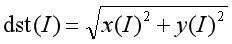# -*- coding: utf-8 -*-
import numpy as np
import cv2
from matplotlib import pyplot as plt

#读取图像

#傅里叶变换
dft = cv2.dft(np.float32(img), flags = cv2.DFT_COMPLEX_OUTPUT)

#将频谱低频从左上角移动至中心位置
dft_shift = np.fft.fftshift(dft)

#频谱图像双通道复数转换为0-255区间
result = 20*np.log(cv2.magnitude(dft_shift[:,:,0], dft_shift[:,:,1]))

#显示图像
plt.subplot(121), plt.imshow(img, cmap = 'gray')
plt.title('Input Image'), plt.xticks([]), plt.yticks([])
plt.subplot(122), plt.imshow(result, cmap = 'gray')
plt.title('Magnitude Spectrum'), plt.xticks([]), plt.yticks([])
plt.show()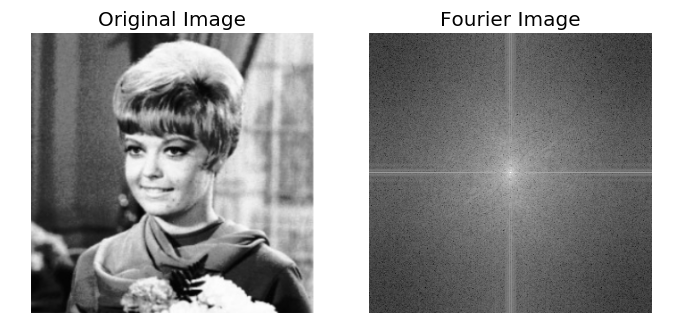# 五.OpenCV实现傅里叶逆变换

dst = cv2.idft(src[, dst[, flags[, nonzeroRows]]])

• src表示输入图像，包括实数或复数
• dst表示输出图像
• flags表示转换标记
• nonzeroRows表示要处理的dst行数，其余行的内容未定义（请参阅dft描述中的卷积示例）

# -*- coding: utf-8 -*-
import numpy as np
import cv2
from matplotlib import pyplot as plt

#读取图像

#傅里叶变换
dft = cv2.dft(np.float32(img), flags = cv2.DFT_COMPLEX_OUTPUT)
dftshift = np.fft.fftshift(dft)
res1= 20*np.log(cv2.magnitude(dftshift[:,:,0], dftshift[:,:,1]))

#傅里叶逆变换
ishift = np.fft.ifftshift(dftshift)
iimg = cv2.idft(ishift)
res2 = cv2.magnitude(iimg[:,:,0], iimg[:,:,1])

#显示图像
plt.subplot(131), plt.imshow(img, 'gray'), plt.title('Original Image')
plt.axis('off')
plt.subplot(132), plt.imshow(res1, 'gray'), plt.title('Fourier Image')
plt.axis('off')
plt.subplot(133), plt.imshow(res2, 'gray'), plt.title('Inverse Fourier Image')
plt.axis('off')
plt.show()# 六.总结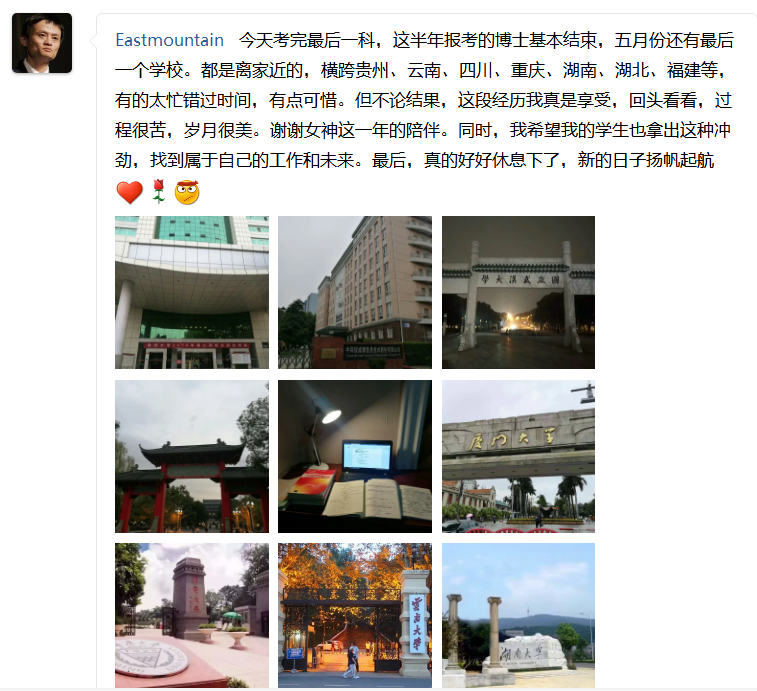（By：Eastmount 2019-04-23 周二下午6点写于花溪 https://blog.csdn.net/Eastmount )

## 毕业课题之------------图像的对称性特征提取

2015-06-09 11:37:07 u012590688 阅读数 1974
• ###### 环路后处理-样点自适应滤波(SAO)

掌握视频编解码的基础知识 讲解新一代视频编解码标准HEVC 通俗易懂 知识点非常全面

104人学习 梅奥
免费试看

依据对称性特征对障碍物进行检测,依照一般定性的分析方法,我们只能知道障碍物是否是对称的,但是在这里仅仅利用对称性的定性分析是不够的,必须能够用数学的形式对其进行定量的描述,下面将对称性的定量分析进行了介绍。本文利用连续对称的概念,建立了定量的方法来度量对称性。图像中的一行灰度数据可视为水平像素坐标的一维函数g(x),(我们不需要知道函数是什么，因为我们最终计算只是用这个函数值，这个类似于核函数的感觉)任何函数都可以写成一个偶函数## 数字图像处理第九章——形态学图像处理

2019-05-11 08:31:12 Dujing2019 阅读数 3570
• ###### 环路后处理-样点自适应滤波(SAO)

掌握视频编解码的基础知识 讲解新一代视频编解码标准HEVC 通俗易懂 知识点非常全面

104人学习 梅奥
免费试看

# 数字图像处理—形态学图像处理

## （一）预备知识

1. 用数学形态学（也称图像代数）表示以形态为基础对图像进行分析的数学工具
2. 基本思想是用具有一定形态的结构元素去度量和提取图像中的对应形状以达到对图像分析识别的目的
3. 形态学图像处理的数学基础和所用语言是集合论
4. 形态学图像处理的应用可以简化图像数据， 保持它们基本的形状特性，并除去不相干的结 构
5. 形态学图像处理的基本运算有4个：膨胀、 腐蚀、开操作和闭操作

#### 1.1 集合理论中的基本概念

1. 所有像素坐标的集合均不属于集合A，记为$A^c$，由下式给出: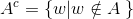这个集合称为集合A的补集

2. 集合B的反射，定义为：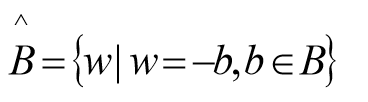即关于原集合原点对称 .

3. 集合A平移到点z=(z1,z2),表示为(A)z，定义为: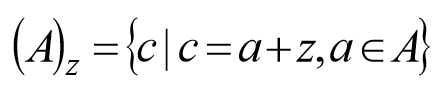#### 1.2 二值图像、集合及逻辑算子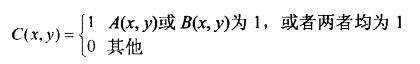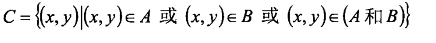1. A为图像集合，B为结构元素（集合）。
2. 数学形态学运算时B对A进行操作。
3. 结构元素要有1个原点（即结构元素参与形态学运算的参考点），可以是中心像素，原则上可选任何像素。
注意：原点可以包含在结构元素中，也可以不包含在结构元素中，但运算的结果常不相同。

f = imread('D:\数字图像处理\第九章学习\Fig0903(a).tif');
subplot(2,3,1), imshow(f);title('(a)二值图像 A:');
subplot(2,3,2), imshow(g);title('(b)二值图像 B:');
subplot(2,3,3), imshow(~f);title('(c)A的补集~A:');
subplot(2,3,4), imshow(f|g);title('(d) A和B的并集 A|B:');
subplot(2,3,5), imshow(f&g);title('(e)A和B的交集 A & B:');
subplot(2,3,6), imshow(f&~g);title('(f)A和B的差集 A&~B');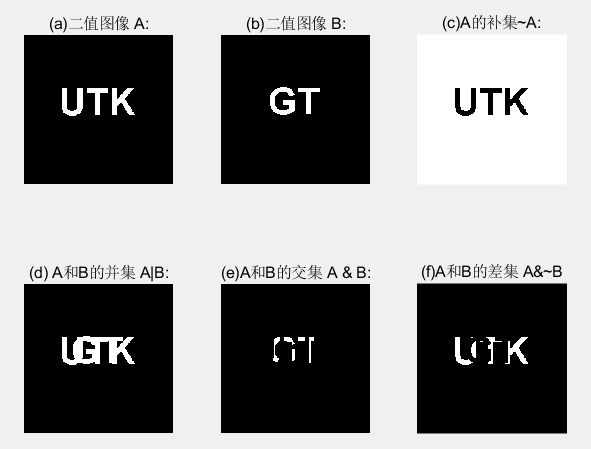## （二）膨胀和腐蚀

#### 2.1 膨胀

A和B是两个集合，A被B膨胀定义为：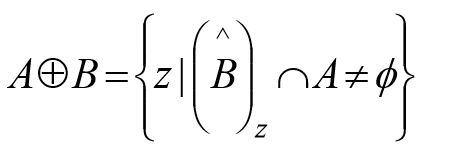1. B的反射进行平移与A的交集不为空。
2. B的反射：相对于自身原点的映象。
3. B的平移：对B的反射进行位移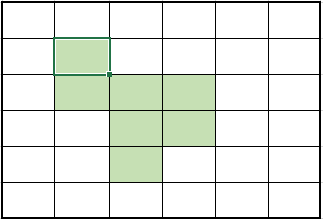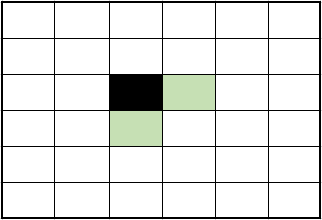(a)集合A    (b)结构元素B (黑色为原点所在)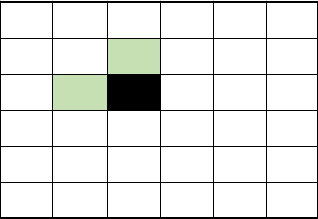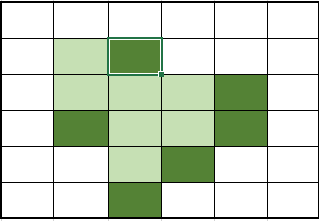(c)结构元素B的映像    (d)图中两种阴影部分（深色为扩大的部分）合起来为A+B

1. 膨胀运算只要求结构元素的原点在目标图像的内部平移，换句话说，当结构元素在目标图像上平移时，允许结构元素中的非原点像素超出目标图像的范围
2. 膨胀运算具有扩大图像和填充图像中比结果元素小的成分的作用，因此在实际应用中可以利用膨胀运算连接相邻物体和填充图像中的小孔和狭窄的缝隙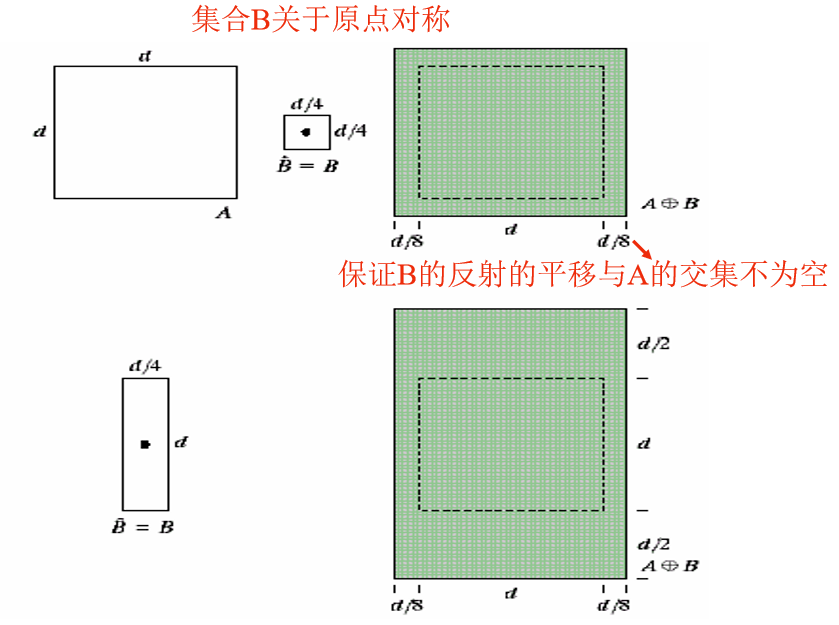D = imdilate(A,B)

A = imread('D:\数字图像处理\第九章学习\Fig0906(a).tif');
B = [0 1 0; 1 1 1; 0 1 0];   %指定结构元素由0和1组成的矩阵
A2 = imdilate(A, B);    %二值图像
subplot(1,2,1), imshow(A);title('(a)包括断开文本的输入图像:');
subplot(1,2,2), imshow(A2);title('(b)膨胀后图像:');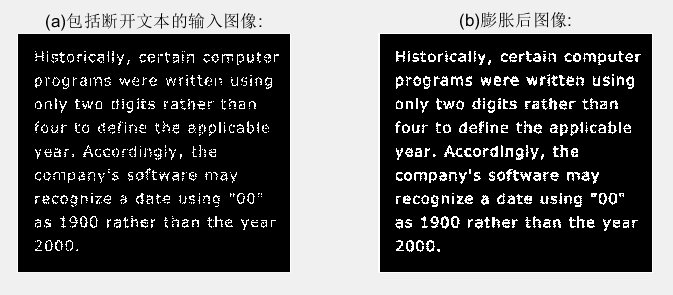#### 2.2 结构元的分解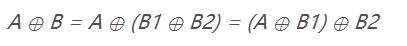B膨胀A等同于B1先膨胀A，再用B2膨胀之前的结果。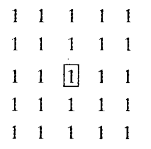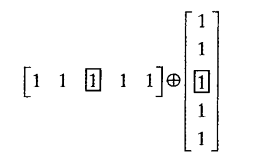#### 2.3 strel函数

se = strel(shape, parameters)

shape用于指定希望形状的字符串，parameters是描述形状信息的参数列表。

#### 2.4 腐蚀

A和B是两个集合，A被B腐蚀定义为：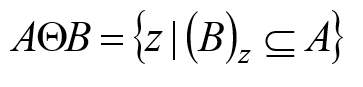1. A被 B 腐蚀是包含在A中的B由z平移的所有点z的集合。
2. B包含在A中的声明相当于B不共享A背景的任何元素。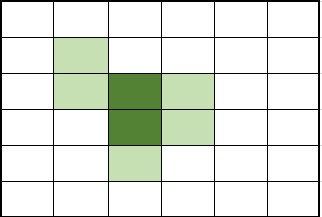(a)集合A(阴影部分)   (b)结构元素B（阴影部分，深色部分为原点）(c)阴影部分合起来为A-B

1. 当结构元素中原点位置不为1（也即原点不属于结构元素时），也要把它看作是1，也就是说，当在目标图像中找与结构元素B相同的子图像时，也要求子图像中与结构元素B的原点对应的那个位置的像素的值是1。
2. 腐蚀运算要求结构元素必须完全包括在被腐蚀图像内部：换句话说，当结构元素在目标图像上平移时，结构元素中的任何元素不能超过目标图像范围。
3. 腐蚀运算的结果不仅与结构元素的形状选取有关，而且还与原点位置的选取有关
4. 腐蚀运算具有缩小图像和消除图像中比结构元素小的成分的作用，因此在实际应用中，可以利用腐蚀运算去除物体之间的粘连，消除图像中的小颗粒噪声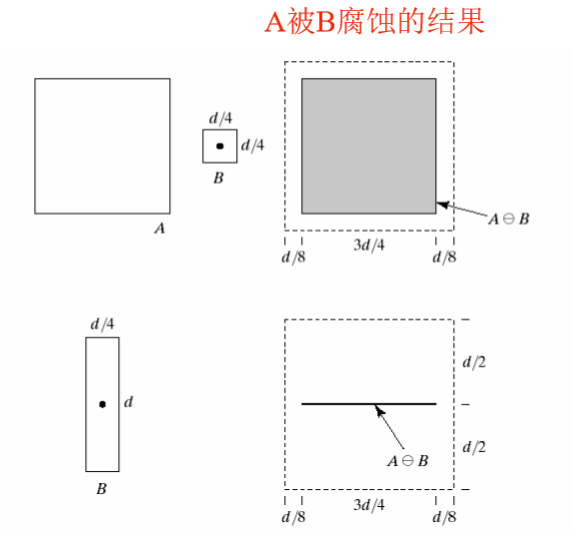A2 = imerode(A, se)

f = imread('D:\数字图像处理\第九章学习\Fig0908(a).tif');
se = strel('disk', 10);
g = imerode(f, se);
se = strel('disk', 5);
g1 = imerode(f, se);
g2 = imerode(f, strel('disk', 20));
subplot(2,2,1), imshow(f);title('(a)原始图像的尺寸为480x480像素:');
subplot(2,2,2), imshow(g);title('(b)用半径为10的圆形腐蚀:');
subplot(2,2,3), imshow(g1);title('(c)用半径为5的圆形腐蚀:');
subplot(2,2,4), imshow(g2);title('(d)用半径为20的圆形腐蚀');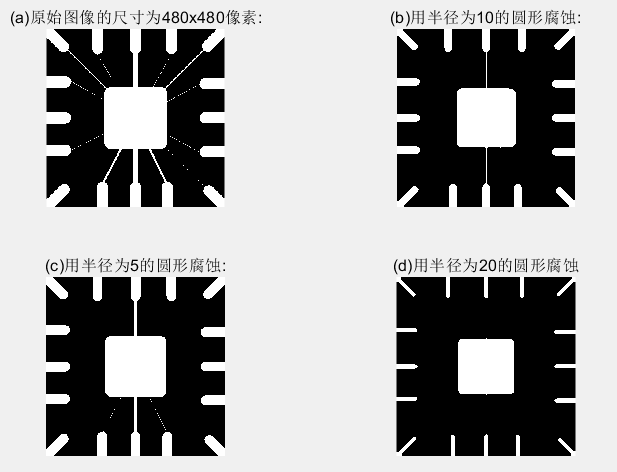## （三） 膨胀与腐蚀的结合

#### 3.1 开操作和闭操作

1. 使图像的轮廓变得光滑，断开狭窄的间断和消除细的突出物。
2. 使用结构元素B对集合A进行开操作，定义为：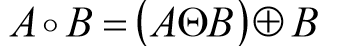先用B对A腐蚀，然后用B对结果膨胀。
3. 与开操作等价的数学表达式为: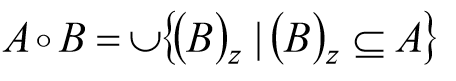4. A o B 的边界通过B中的点完成。
5. B在A的边界内转动时，B中的点所能到达的A的边界的最远点。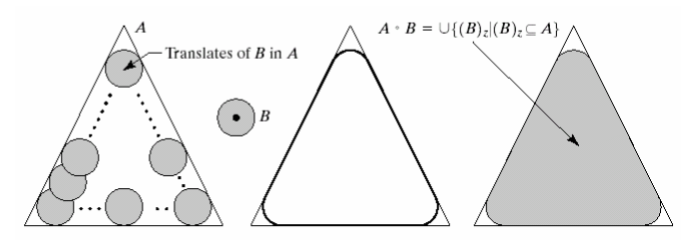6. A o B 是 A的子集合。
7. 如果C是D的子集，则 C o B是 D o B的子集。
8. (A o B) o B = A o B

1. 同样使图像的轮廓变得光滑，但与开操作相反，它能消除狭窄的间断和长细的鸿沟，消除小的孔洞，并填补轮廓线中的裂痕。
2. 使用结构元素B对集合A进行闭操作，定 义为：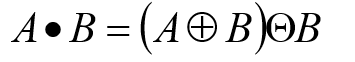先用B对A膨胀，然后用B对结果腐蚀。
3. A . B的边界通过B中的点完成 。
4. B在A的边界外部转动 :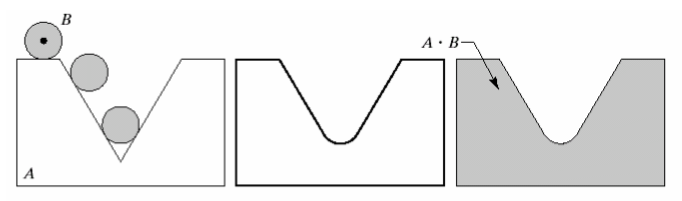5. A 是 A . B的子集合。
6. 如果C 是 D 的子集 ， 则C . B 是 D . B的子集。
7. (A . B) . B = A . B

C = imopen(A, B)

C = imclose(A, B)

A为二值图像，B为0，1矩阵组成，并且是指定结构元素。

f = imread('D:\数字图像处理\第九章学习\Fig0910(a).tif');
se = strel('square', 40);
fo = imopen(f, se);
fc = imclose(f, se);
foc = imclose(fo, se);
subplot(2,2,1), imshow(f), title('(a)原图');
subplot(2,2,2), imshow(fo), title('(b)开操作');
subplot(2,2,3), imshow(fc), title('(c)闭操作');
subplot(2,2,4), imshow(foc), title('(d) (b)的闭操作结果');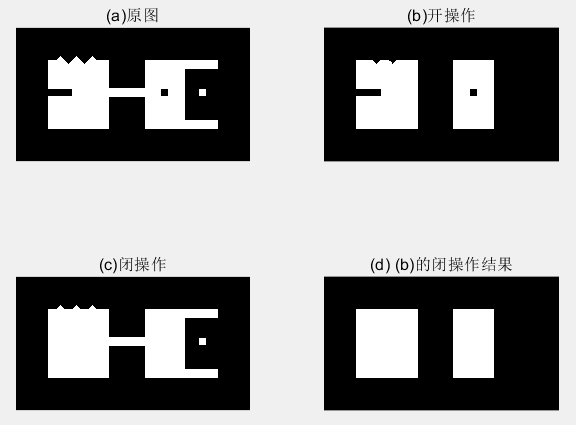1. 图(a)中的图像设计了一些用于演示开操作和闭操作的特征，比如细小突起、细的桥接点、几个弯口、孤立的小洞、 小的孤立物和齿状边缘。
2. 图 (b)显示了结果。注意，从图中可以看出，细的突出和外部点的边缘的不规则部分被去除掉了，细的桥接和小的孤立物也被去除了。
4. 图 (d)显示了平滑过的物体。

f = imread('D:\数字图像处理\第九章学习\Fig0911(a).tif');
se = strel('square', 6);
fo = imopen(f, se);
foc = imclose(fo, se);
subplot(1,3,1), imshow(f), title('(a)带噪声的指纹图像');
subplot(1,3,2), imshow(fo), title('(b)图像的开操作');
subplot(1,3,3), imshow(foc), title('(c)先用开操作，再用闭操作');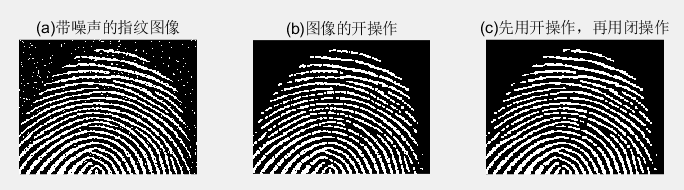1. 图(a)是受噪声污染的指纹二值图像，噪声为黑色背景上的亮元素和亮指纹部分的暗元素。
2. 图(b)所示的图像。发现，对图像进行开操作可以去除噪声点，但是这种处理在指纹的纹脊上又引入一些缺口
3. 图( c )显示了最终结果。在这个结果中，大多数噪声被消除了，开运算的闭运算可以给指纹填充缺口，但是指纹纹路并没有完全恢复 。

#### 3.2 击中或击不中变换

A中对B进行的匹配（击中）表示为：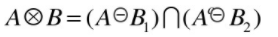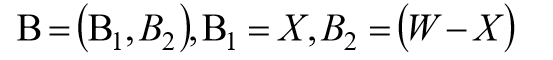B1是由与一个对象相联系的B元素构成的集合，B1是由与一个对象相联系的B元素构成的集合。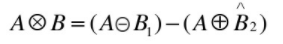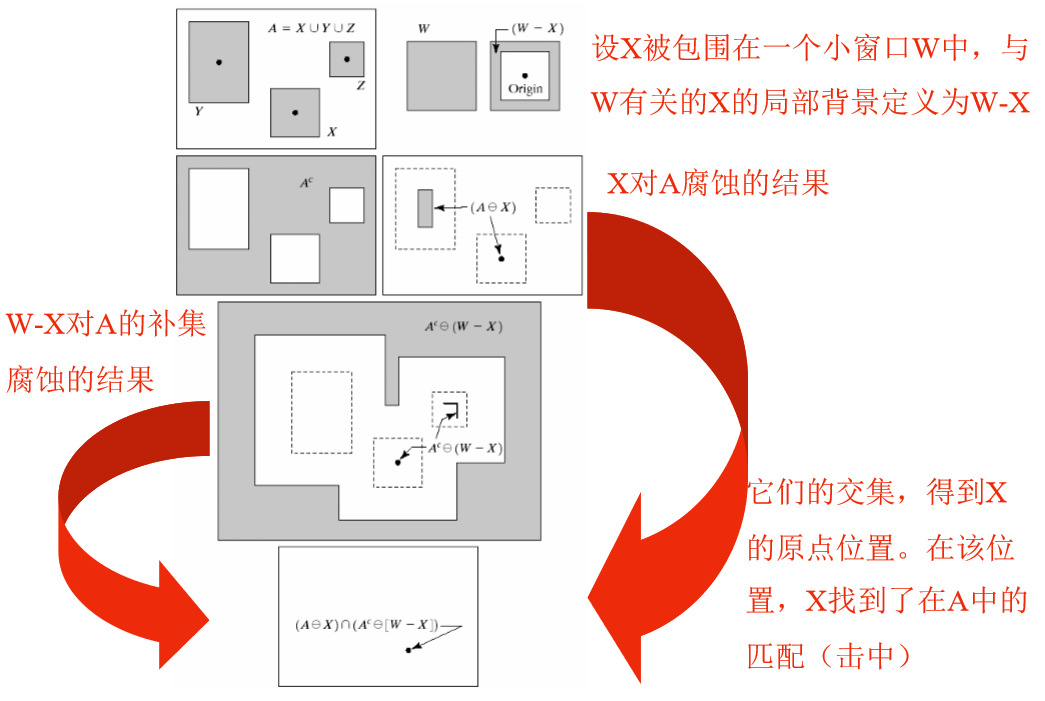C = bwhitmiss(A, B1, B2)

f = imread('D:\数字图像处理\第九章学习\Fig0913(a).tif');
B1 = strel([0 0 0;0 1 1; 0 1 0]);
B2 = strel([1 1 1;1 0 0;1 0 0]);
g = bwhitmiss(f,B1,B2);
subplot(1,2,1), imshow(f), title('(a)原始图像');
subplot(1,2,2), imshow(g), title('(b)击中、击不中变换的结果');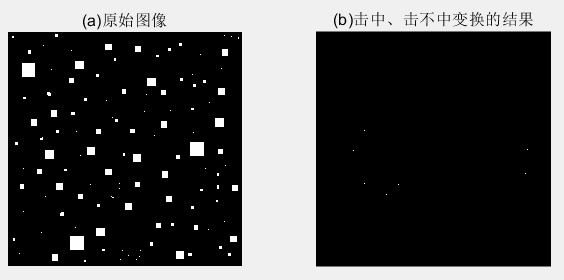1. 图(a)显示了包括各种尺寸的正方形图像。我们要定位有东、南相邻像素(这些 “击中”）和没有东北、北、西北、西和西南相邻像素(这些 “击不中”）的前景像素。这些要求导致以下B1,B2两个结构元。这两个结构元都不包括东南邻域像素，这称为不关心像素。用函数 bwhitmiss 来计算变换。
2. 图 (b)中的每个单像素点都是图 (a)中物体左上角的像素。图 (b)中是放大后的像素，以便更清晰。bwhitmiss的替代语法可以把Bl 和 B2 组合成间隔矩阵。只要 B1等于 1 或-1，B2 等于 1, 间隔矩阵就等于 1。对于不关心像素，间隔矩阵等于 0。

#### 3.3 bwmorph函数

g = bwmorph(f, operation, n);

f 是输入的二值图像，operation 是指定所希望运算的字符串，n 是指定重复次数的正整数。

f = imread('D:\数字图像处理\第九章学习\Fig0911(a).tif');
g1 = bwmorph(f, 'thin',1);
g2 = bwmorph(f, 'thin',2);
ginf = bwmorph(f,'thin', Inf);
subplot(1,4,1),imshow(f);title('(a)指纹图像:');
subplot(1,4,2),imshow(g1);title('(b)细化一次后的指纹图像:');
subplot(1,4,3),imshow(g2);title('(c)细化两次后的图像:');
subplot(1,4,4),imshow(ginf);title('(d)一直细化到稳定状态的图像：');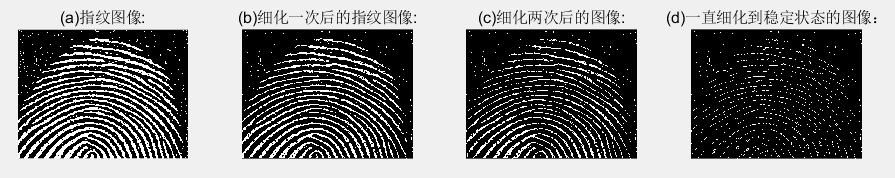f = imread('D:\数字图像处理\第九章学习\Fig0916(a).tif');
fs = bwmorph(f,'skel',Inf);
for k = 1:5
fa = fs & ~endpoints(fs);
end
subplot(1,3,1),imshow(f);title('(a)骨头图像:');
subplot(1,3,2),imshow(fs);title('(b)使用函数 bwmorph 得到的骨豁:');
subplot(1,3,3),imshow(fa);title('（c)使用函数 endpoint 裁剪后的骨豁：');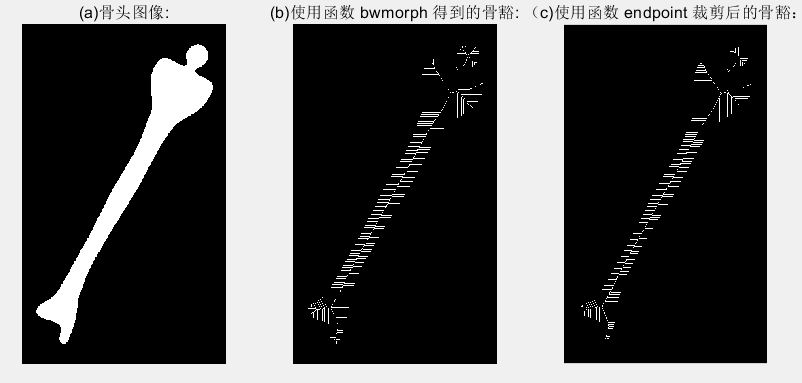## （四）标记连通分量

[L, num] = bwlabel (f, conn)

f 是输入二值图像，coon指定希望的连接方式（不是4连接就是8连接），输出L叫做标记矩阵，函数num则给出找到的连通分量总数。

f = imread('D:\数字图像处理\第九章学习\Fig0917(a).tif');
imshow(f);title('(a)标注连通分量原始图像:');
[L,n]=bwlabel(f);        %L为标记矩阵，n为找到连接分量的总数
[r,c]=find(L==3);        %返回第3个对象所有像素的行索引和列索引
rbar=mean(r);
cbar=mean(c);
figure,imshow(f);title('(b)标记所有对象质心后的图像:');
hold on            %保持当前图像使其不被刷新
for k=1:n
[r,c]=find(L==k);
rbar=mean(r);
cbar=mean(c);
plot(cbar,rbar,'Marker','o','MarkerEdgeColor','k',...
'MarkerFaceColor','k','MarkerSize',10);
plot(cbar,rbar,'Marker','*','MarkerFaceColor','w'); %其中的marker为标记
end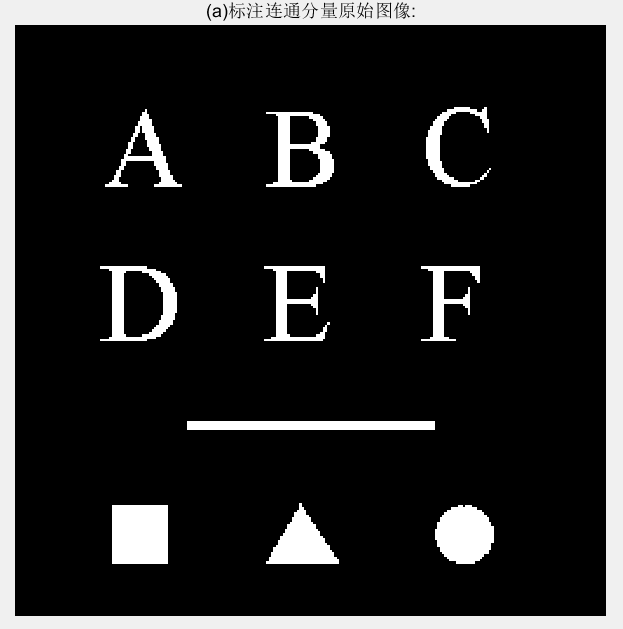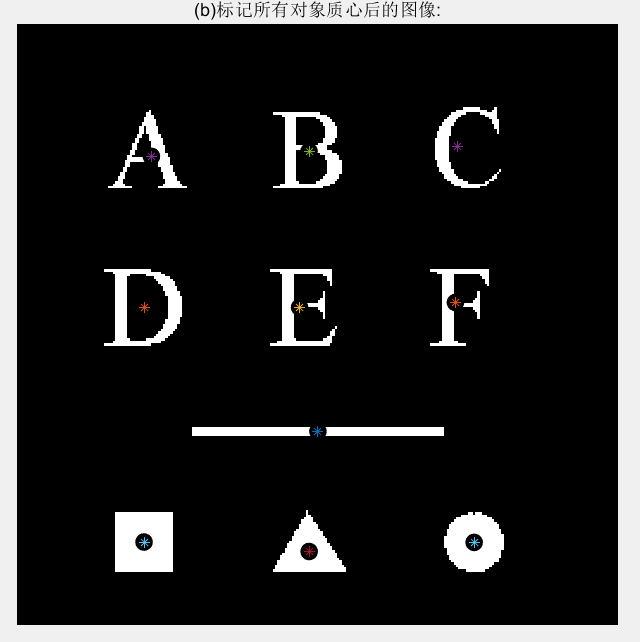## （五）形态学重建

1. 将h1初始化为标记图像f。
2. 创建结构元素 ：B = ones(3)。
3. 重复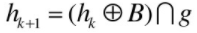直到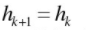其中，标记f必须是g的一个子集。

#### 5.1 通过重建进行开操作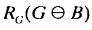f = imread('D:\数字图像处理\第九章学习\Fig0917(a).tif');
subplot(3,2,1),imshow(f);title('(a)重构原始图像');
fe=imerode(f,ones(51,1));%竖线腐蚀
subplot(3,2,2),imshow(fe);title('(b)使用竖线腐蚀后的结果');
fo=imopen(f,ones(51,1));%竖线做开运算
subplot(3,2,3),imshow(fo);title('(c)使用竖线做开运算结果');
fobr=imreconstruct(fe,f);%fe做标记
subplot(3,2,4),imshow(fobr);title('(d)使用竖线做重构开运算');
ff=imfill(f,'holes');%对f进行孔洞填充
subplot(3,2,5),imshow(ff);title('(e)对f填充孔洞后的图像');
fc=imclearborder(f,8);%清除边界，2维8邻接
subplot(3,2,6),imshow(fc);title('(f)对f清除边界后的图像');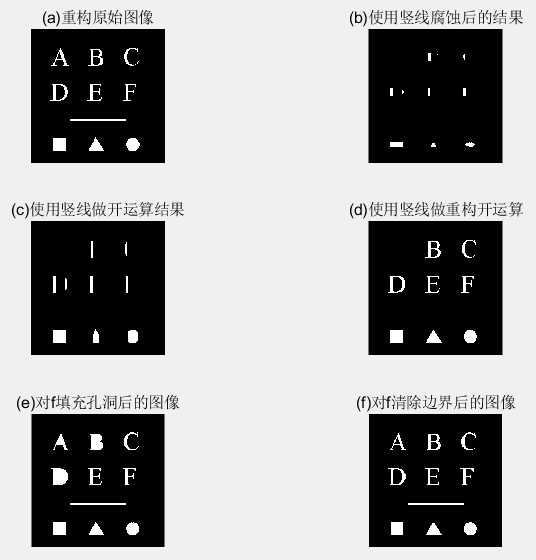1. 传统开运算中，腐蚀去除掉小对象，随后的膨胀恢复原始对象形状，但受元素结构影响，恢复的往往不是很精确。
2. 重构则能精确恢复原始图像。

#### 5.2 填充孔洞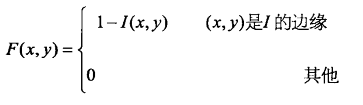g = imfill(f,‘holes’);

#### 5.3 清除边界物体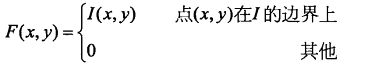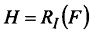g = imclearborder(f,conn)

f 是输入图像，g 是结果。conn 的值不是 4 就是 8(默认)。 物体更亮且与图像边界相连接的结构。

## （六）灰度级形态学

#### 6.1 膨胀和腐蚀

1. 使用结构元素b对f的灰度膨胀定义为：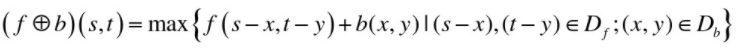其中，$D_f$$D_b$分别是f和b的定义域，f和b是函数而不是二值形态学情况中的集合。

2. 当结构元素b是平坦的，即b(x,y)在其定义域内都为0时：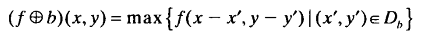1. 使用结构元素b对f的灰度腐蚀定义为：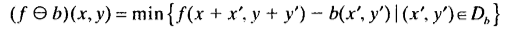其中，$D_f$$D_b$分别是f和b的定义域。

2. 当结构元素b是平坦的，即b(x,y)在其定义域内都为0时：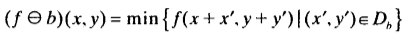f = imread('D:\数字图像处理\第九章学习\Fig0923(a).tif');
se=strel('square',3);  %构造了一个平坦的3x3的结构元素
gd=imdilate(f,se);    %对原图像进行膨胀操作
ge=imerode(f,se);     %对原图像进行腐蚀操作
subplot(3,2,1);imshow(f,[]);title('(a)原始图像');
subplot(3,2,2),imshow(gd,[]);title('(b)膨胀的图像');
subplot(3,2,3),imshow(ge,[]);title('(c)腐蚀的图像');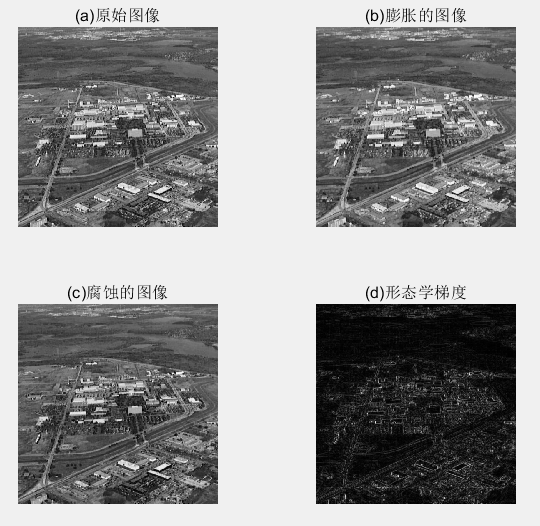1. 膨胀得到的图像比原图像更明亮，并且减弱或消除小的，暗的细节部分。即比原图像模糊。
2. 腐蚀得到的图像更暗，并且尺寸小，明亮的部分被削弱 。

#### 6.2 开操作和闭操作

1. 在灰度图像中，开操作的表达式与二值图像拥有相同的形式。2. 把一幅图像看做是一个三维表明，其亮度值代表xy平面上的高度值，则当结构元素b在f下面活动时，结构元素的任何部分的最高值构成了开运算的结果。
3. 先进行腐蚀操作可以除去小的亮的图像细节，但这样会使图像变暗，接下来进行膨胀操作增强图像的整体亮度。

1. 在灰度图像中，闭操作的表达式与二值图像拥有相同的形式。2. 当结构元素b在f的上面活动时，结构元素的任何部分的最低值构成了闭运算的结果 。
3. 先通过膨胀操作除去图像中的暗细节，同时增加图像的亮度，接下来对图像进行腐蚀，而不会将膨胀操作除去的部分重新引入图像中。

f = imread('D:\数字图像处理\第九章学习\Fig0925(a).tif');
subplot(3,2,1),imshow(f);
title('(a)木钉图像原图');
se=strel('disk',5);     %disk其实就是一个八边形
fo=imopen(f,se);        %经过开运算
subplot(3,2,2),imshow(f);
title('(b)使用半径5的disk开运算后的图像');
foc=imclose(fo,se);
subplot(3,2,3),imshow(foc);
title('(c)先开后闭的图像');
focd=imclose(f,se);
subplot(3,2,4),imshow(focd);
title('(d)原始图像的闭操作');
foce=imopen(focd,se);
subplot(3,2,5),imshow(foce);
title('(e)先闭后开的图像');
fasf=f;
for i=2:5
se=strel('disk',i);
fasf=imclose(imopen(fasf,se),se);
end
subplot(3,2,6),imshow(fasf);
title('(f)使用开闭交替滤波后图像');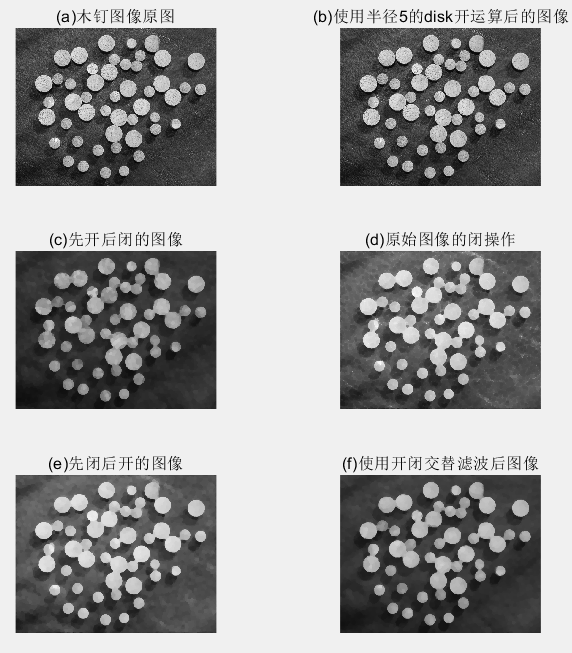1. 图 (b)显示了开操作的图像 fo, 在这里，我们看到，亮区域己经被调低了(平滑)，木钉上的暗条文几乎没有受影响。
2. 图 (c )显示了开操作的闭操作 foe。现在我们注意到，暗区域已经被平滑得很好了，结果是整个图像得到全部平滑。这种过程通常叫做开-闭滤波。先开运算后闭运算构成噪声滤波器，用来平滑图像并去除噪声。
3. 图 (d)显示了原始图像的闭操作结果。木钉上的暗条文已经被平滑掉了，主要留下了亮的细节(注意背景中的亮条文)。
4. 图 (e)显示了这些条文的平滑和木钉表面的进一步平滑效果。最终结果是原始图像得到全部平滑。

f = imread('D:\数字图像处理\第九章学习\Fig0926(a).tif');
g = f>=(255*graythresh(f));
se=strel('disk',100);
fo=imopen(f,se);
f2=imsubtract(f,fo);
g1 = f2>=(255*graythresh(f2));
subplot(2,3,1),imshow(f);
title('(a)原始图像');
subplot(2,3,2),imshow(g);
title('(b)经过阈值处理后的图像');
subplot(2,3,3),imshow(f);
title('(c)原图开运算后的图像');
subplot(2,3,4),imshow(f2);
title('(d)原图减去开运算');
subplot(2,3,5),imshow(g1);
title('(e)最终结果');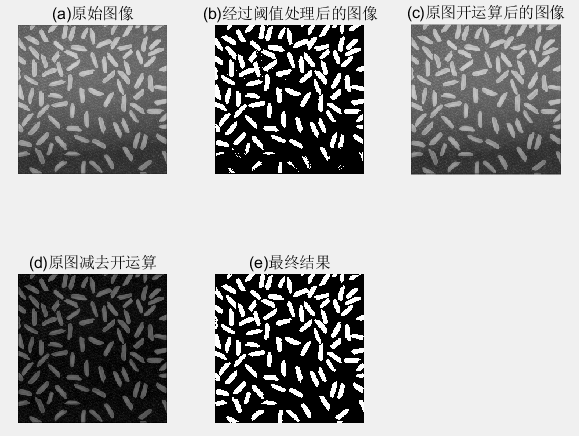1. 图 (a) :显示了一幅米粒的图像f，图像下部的背景比上部的黑。这样的话，对不平坦的亮度进行阈值处理会很困难。
2. 图 (b) "是阈值处理方案，图像顶端的米粒被很好地从背景中分离开来，但是图像底部的米粒没有从背景中正确地提取出来。
3. 图(c ):对图像进行开操作，可以产生对整个图像背景的合理估计。
4. 图(d) :把图(c )从原始图像中减去，生成一幅拥有合适的均勾背景的米粒图像.
5. 图(e):显示了新的经阈值处理后的图像。注意，改进效果超过了图 (b)。

f = imread('D:\数字图像处理\第九章学习\Fig0926(a).tif');
sumpixels=zeros(1,36);
for k=0:35
se=strel('disk',k);
fo=imopen(f,se);
sumpixels(k+1)=sum(fo(:));
end
%可以看到，连续开运算之间的表面积会减少
plot(0:35,sumpixels),xlabel('k'),ylabel('surface area');
title('(a)表面积和结构元素半径之间的关系');
figure,plot(-diff(sumpixels));%diff()函数为差分或者近似倒数，即相邻2个之间的差值
xlabel('k'),ylabel('surface area reduction');
title('(b)减少的表面积和结构元素半径之间的关系');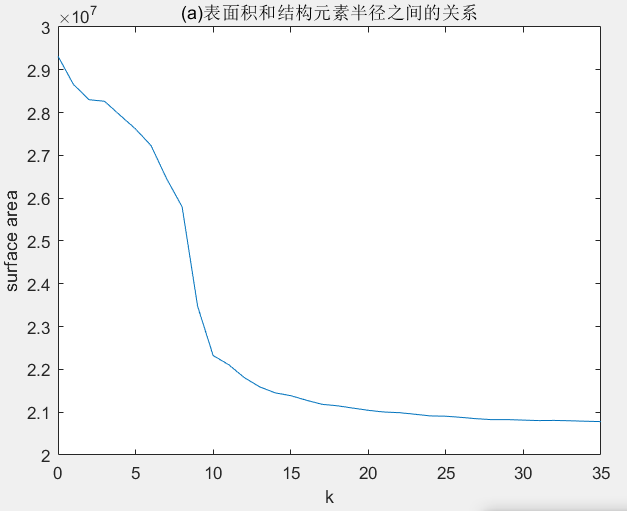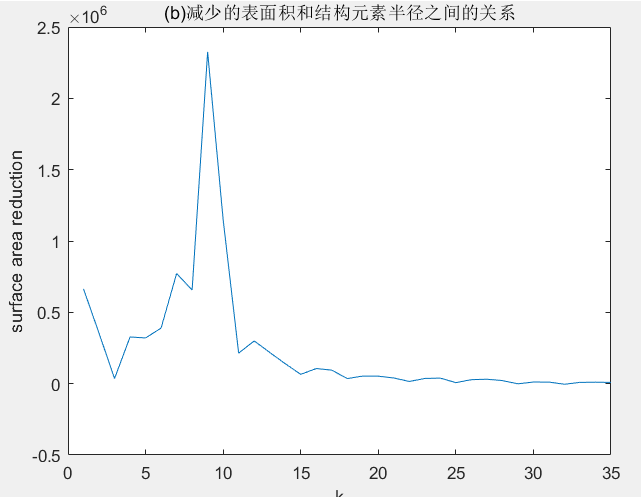1. (a)连续开运算之间的表面积会减小。
2. (b)图峰值表明出现了大量的有着这种半径的对象。

#### 6.3 重建

1. h极小值变换：标记图像是由掩膜挑选ing减去常量所得。
2. 开运算重建：先腐蚀后重建。
3. 闭运算重建：对图像求补、计算其开操作重建并对结果求补。

f = imread('D:\数字图像处理\第九章学习\Fig0930(a).tif');
subplot(3,3,1),imshow(f);
title('(a)原图像');
f_obr=imreconstruct(imerode(f,ones(1,71)),f);
subplot(3,3,2),imshow(f_obr);
title('(b)重建的开操作');
f_o=imopen(f,ones(1,71));
subplot(3,3,3),imshow(f_o);
title('(c)开操作');
f_thr=imsubtract(f,f_obr);    %顶帽重构
subplot(3,3,4),imshow(f_thr);
title('(d)重建的顶帽操作');
f_th=imsubtract(f,f_o)    %标准顶帽运算，方便比较
subplot(3,3,5),imshow(f_th);
title('(e)顶帽操作');
g_obr=imreconstruct(imerode(f_thr,ones(1,11)),f_thr);
subplot(3,3,6),imshow(g_obr);
title('(f)用水平线对(b)经开运算后重建图');
g_obrd=imdilate(g_obr,ones(1,2));
subplot(3,3,7),imshow(g_obrd);
title('(g)使用水平线对(f)进行膨胀');
f2=imreconstruct(min(g_obrd,f_thr),f_thr);
subplot(3,3,8),imshow(f2);
title('(h)最后的重建结果');